• ## matlab画三维图像

千次阅读 2018-08-14 11:11:43
数学学习中，有很多地方需要画图来直观对比显示，本节记录一下用matlab画三维图形的几种方法。 例：, 1、surf、surfc、surfl surf:   clear clc close all %% gride [x,y]=meshgrid(0:0.1:3,0:0.1:3); %% ...
数学学习中，有很多地方需要画图来直观对比显示，本节记录一下用matlab画三维图形的几种方法。

例：,

1、surf、surfc、surfl

surf:

clear
clc
close all
%% gride
[x,y]=meshgrid(0:0.1:3,0:0.1:3);

%% plot
z = x.^2-y;
surf(x,y,z)
xlabel('X')
ylabel('Y')
zlabel('Z')
title('z=x^2-y')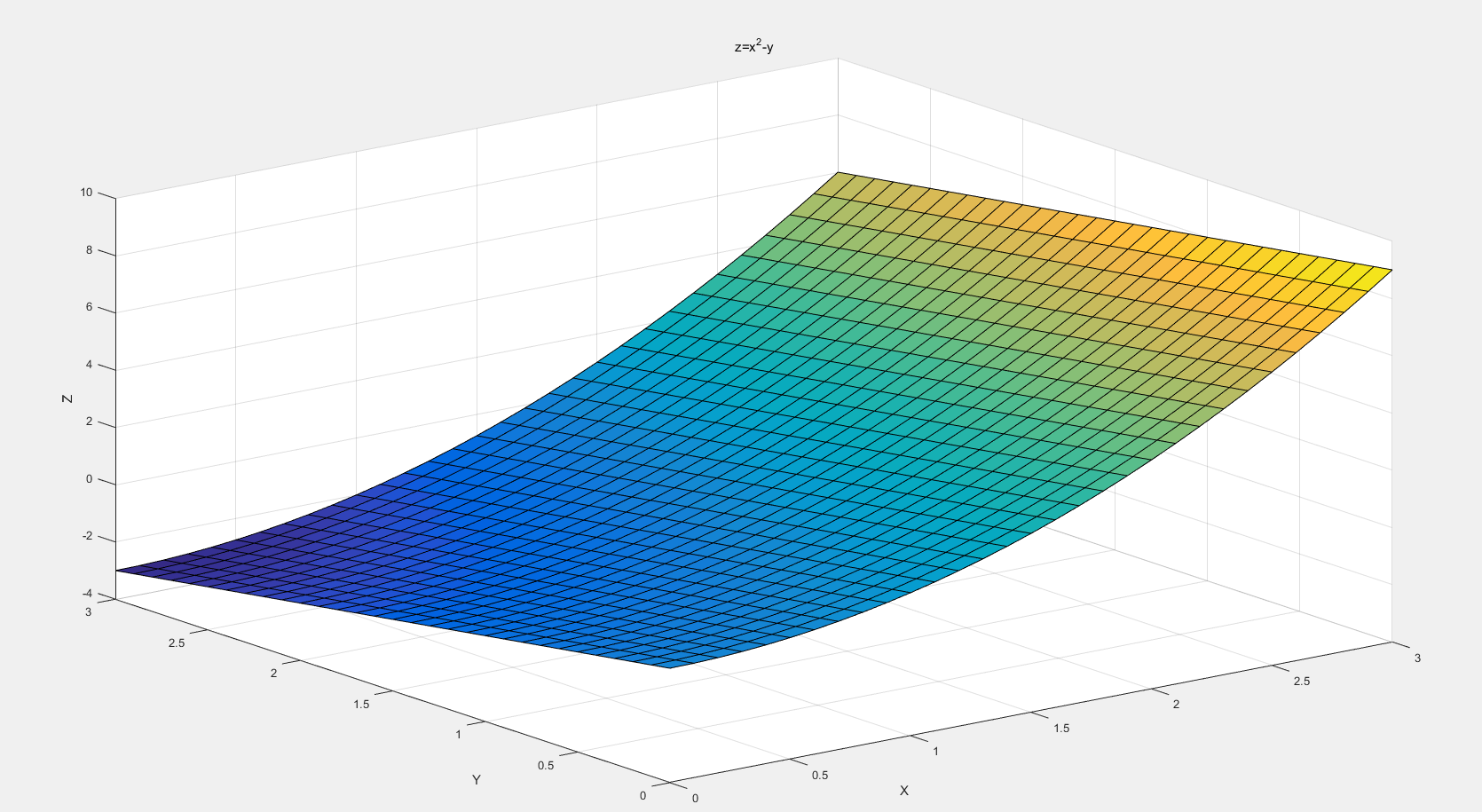surfc：有等高线

surfc(x,y,z)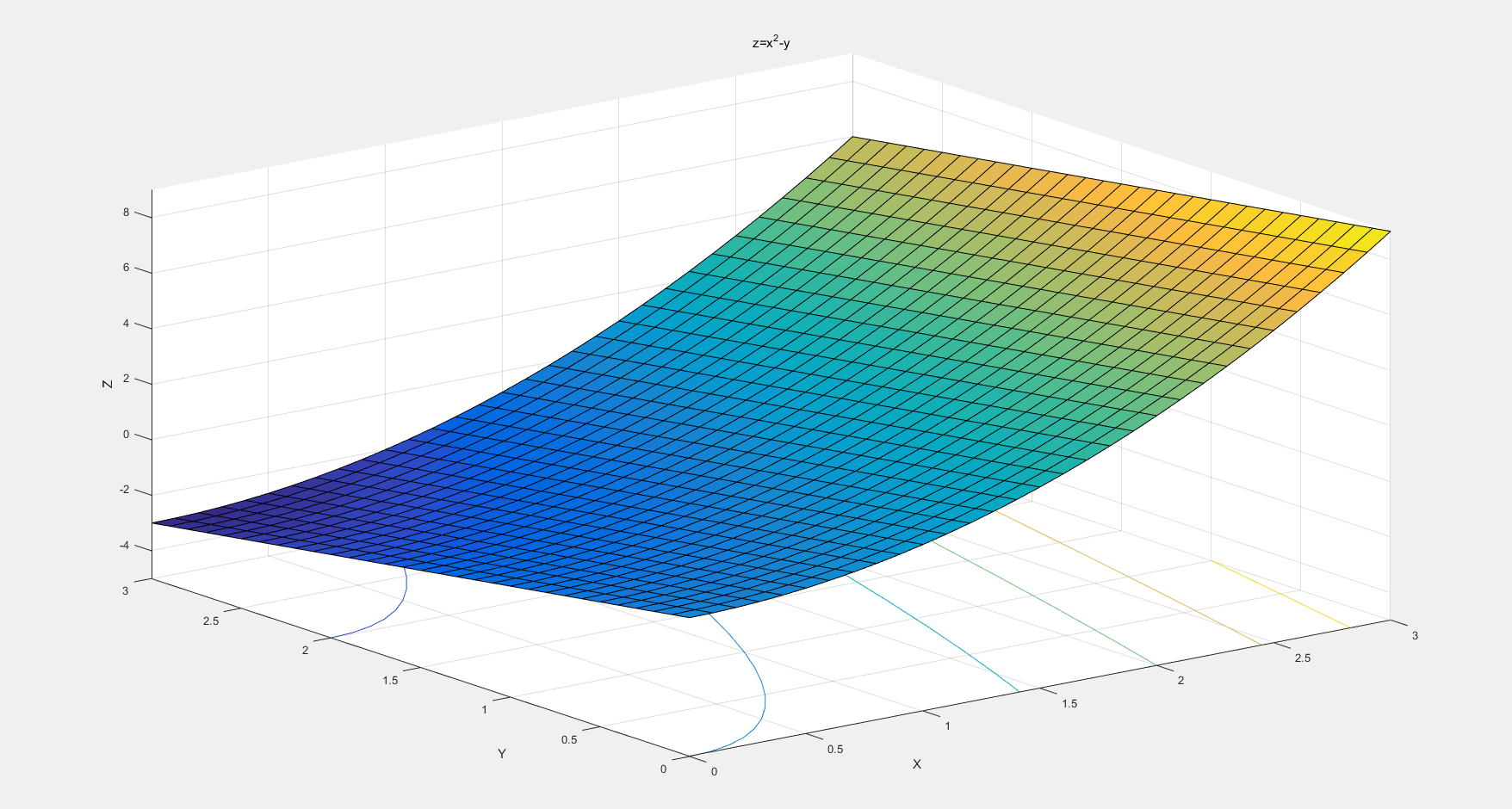surfl：MATLAB中内置了surfl函数，基于运用漫反射、镜面发射光和环境照明模型。使用一个单色颜色映像（如灰色，纯白，铜黄或粉红色）和插值色彩，会画出效果更好的曲面

surfl(x,y,z,s)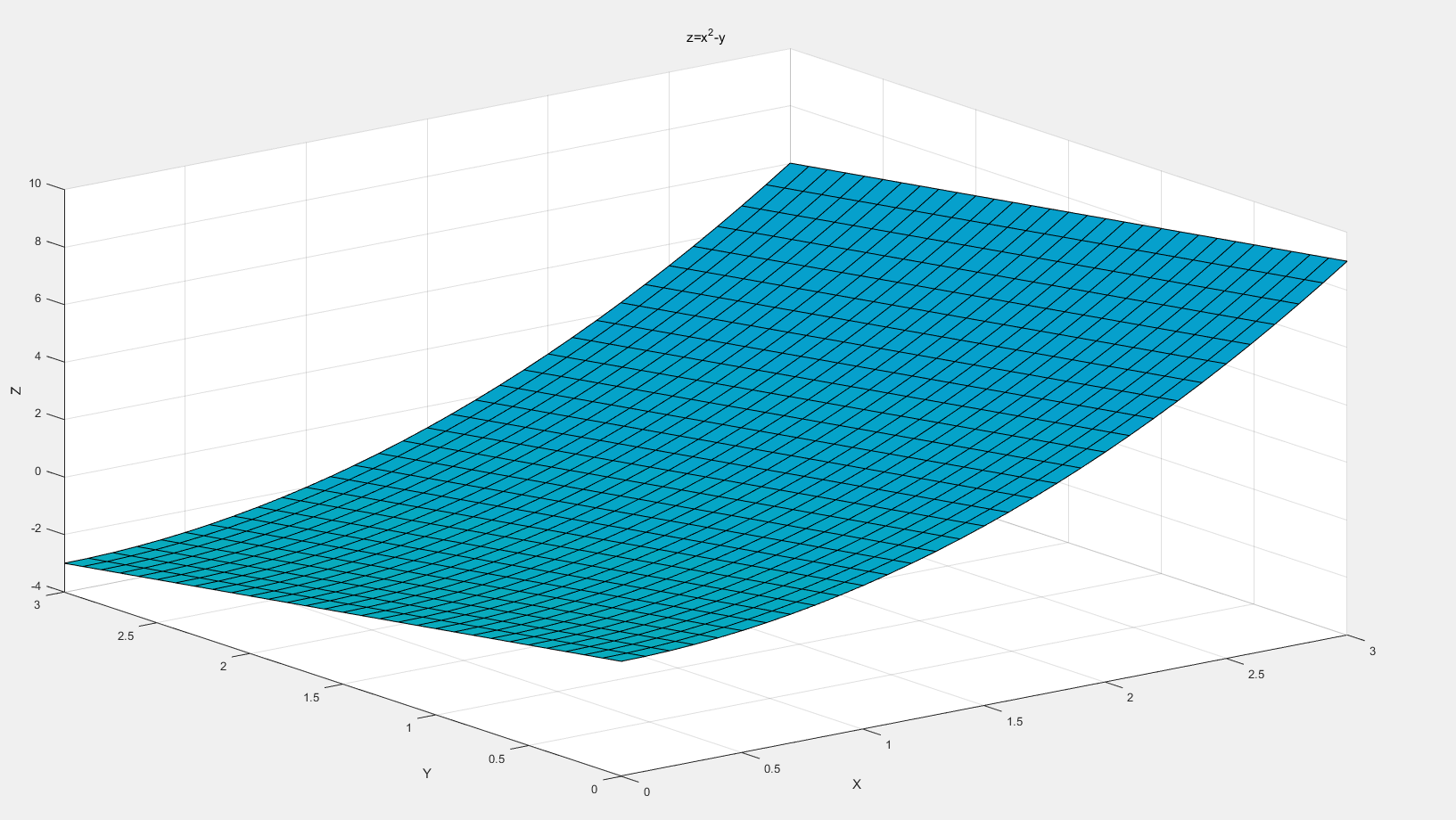2、mesh

clear
clc
close all
%% grid
[x,y]=meshgrid(0:0.1:3,0:0.1:3);

%% first
% plot
z = x.^2-y;
c=zeros(31,31);
c=c+0.1;
mesh(x,y,z,c)
xlabel('X')
ylabel('Y')
zlabel('Z')
% make label
hold on
s='x^2-y';
text(x(1,31),y(1,31),z(1,31),s,'fontsize',15,'HorizontalAlignment','center');

%% second
% plot
hold on
z1=sqrt(x)-y;
c1=c+0.2;
mesh(x,y,z1,c1)
% make label
hold on
s='√x-y';
text(x(1,31),y(1,31),z1(1,31),s,'fontsize',15,'HorizontalAlignment','center');

%% third
% plot
hold on
z2=sqrt(x+y)-2*x;
c2=c+0.3;
mesh(x,y,z2,c2)
% make lable
hold on
s='√(x+y)-2y';
text(x(1,31),y(1,31),z2(1,31),s,'fontsize',15,'HorizontalAlignment','center');

title('x^2-y,√x-y,√(x+y)-2y')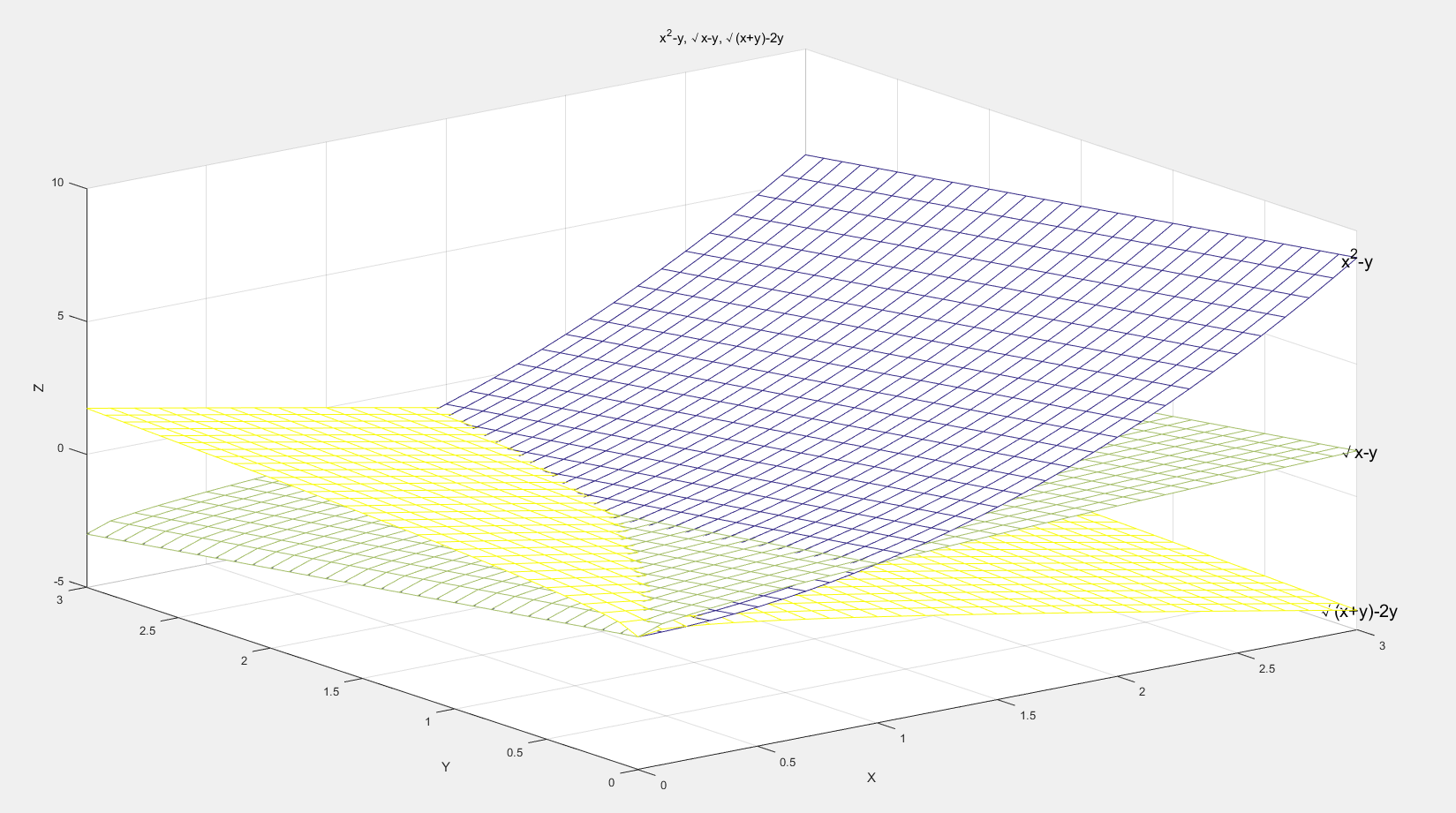展开全文•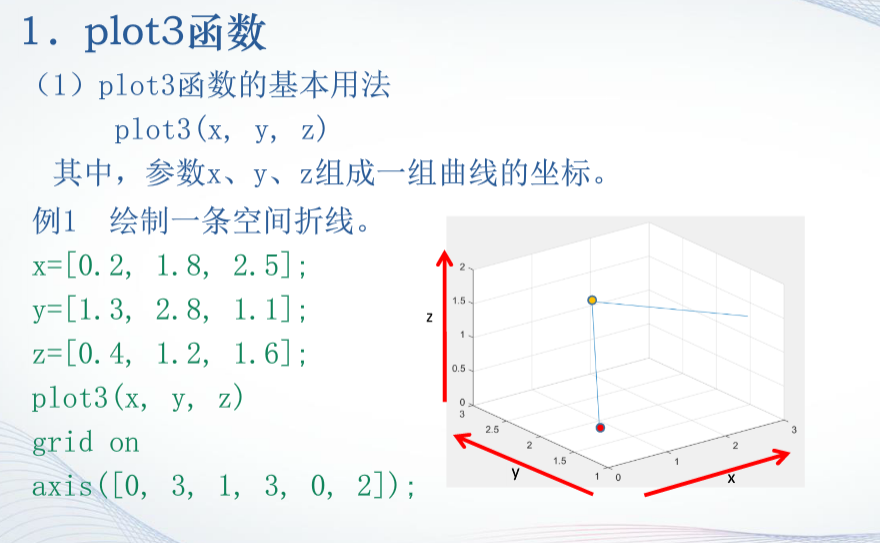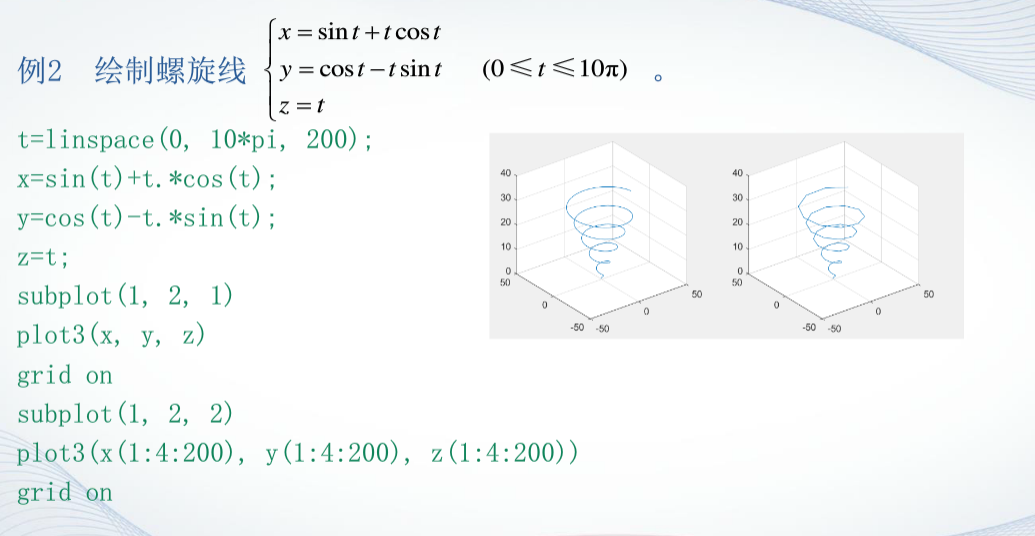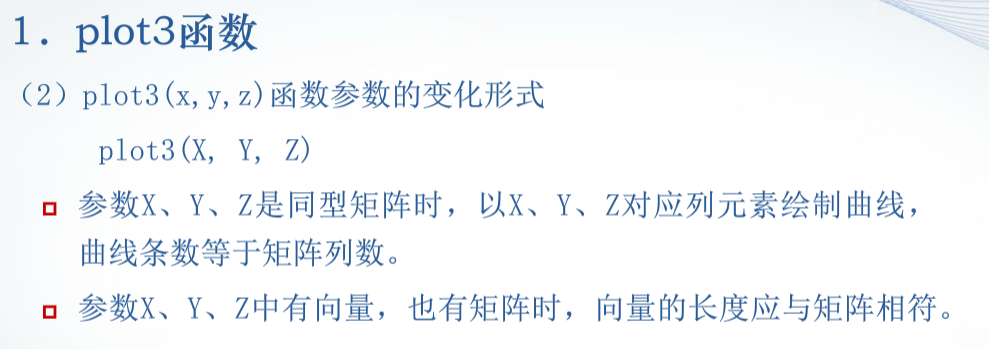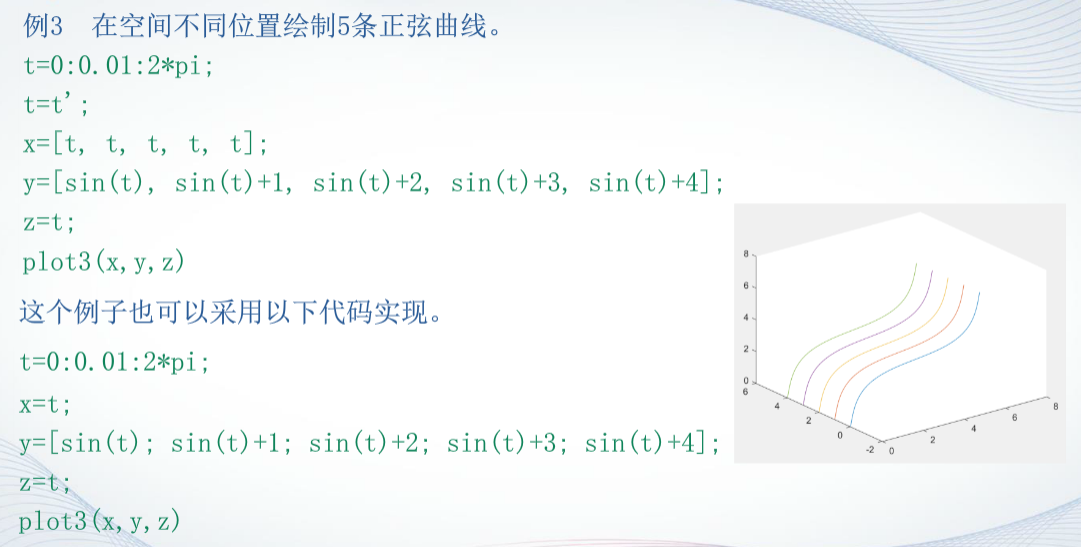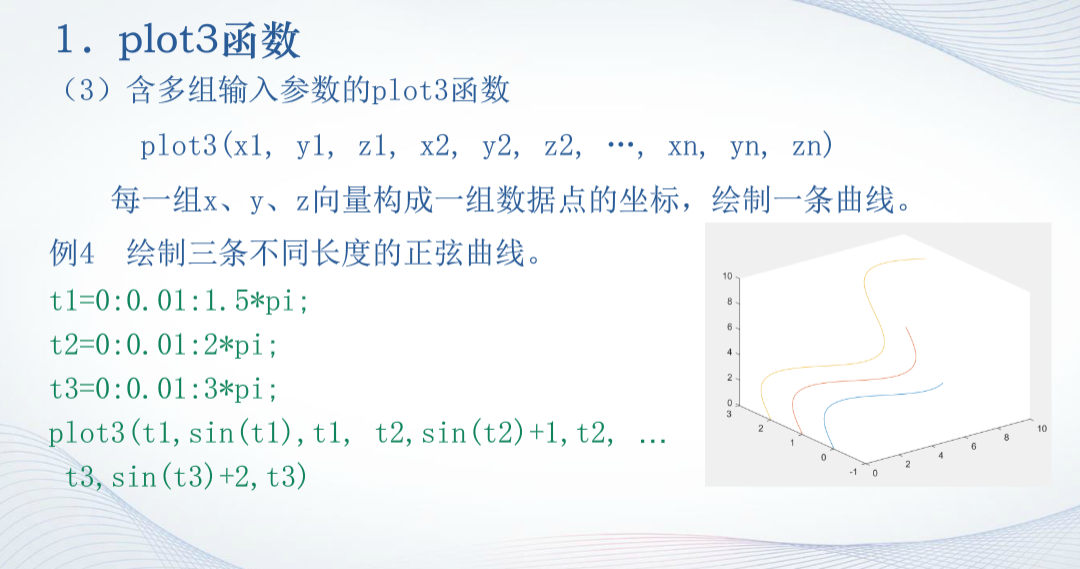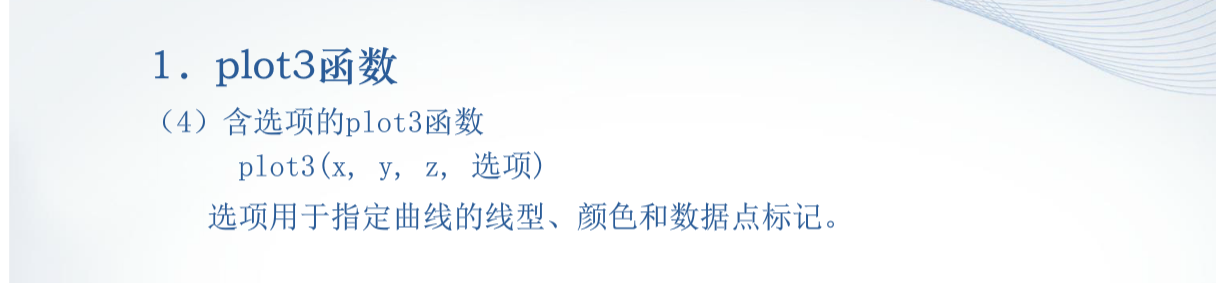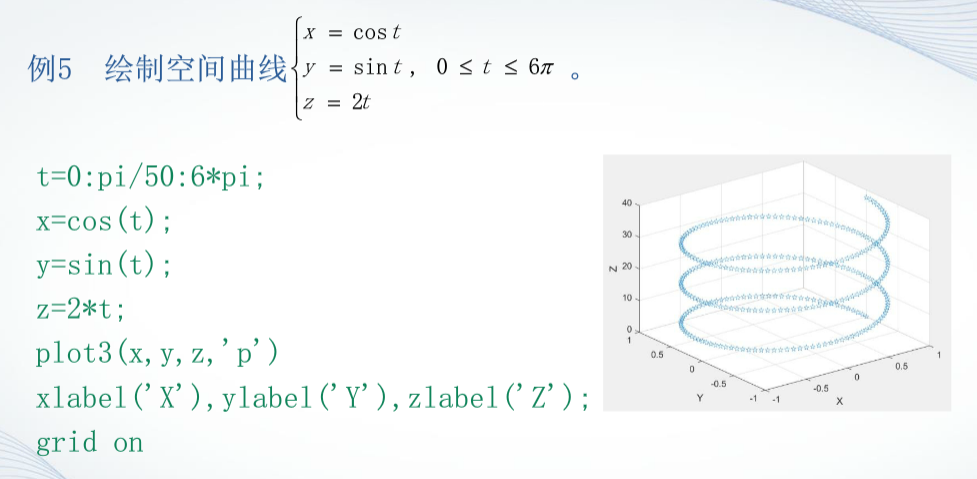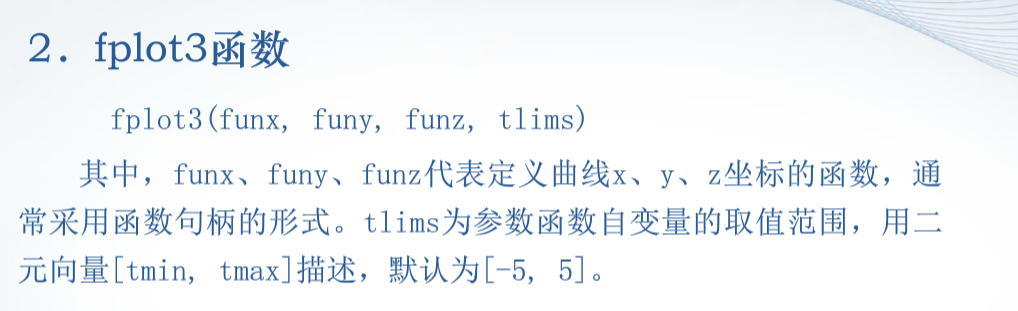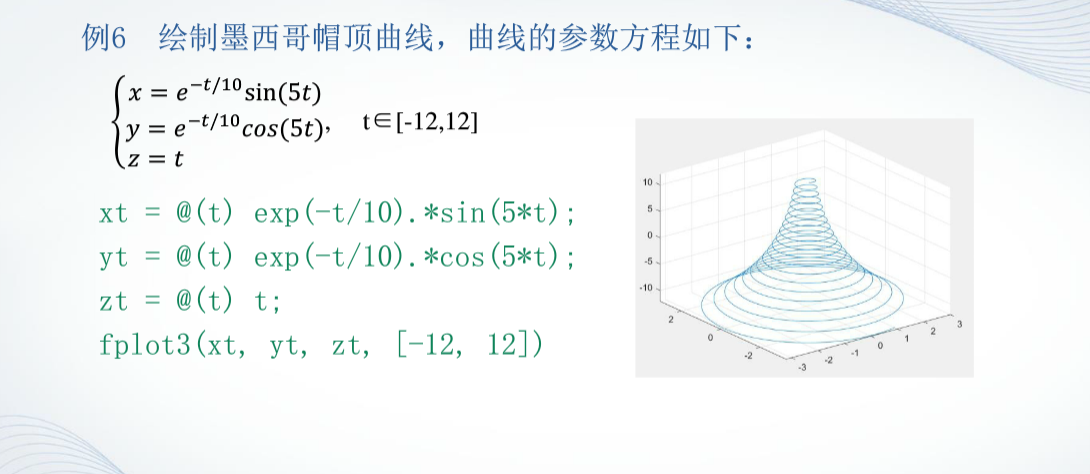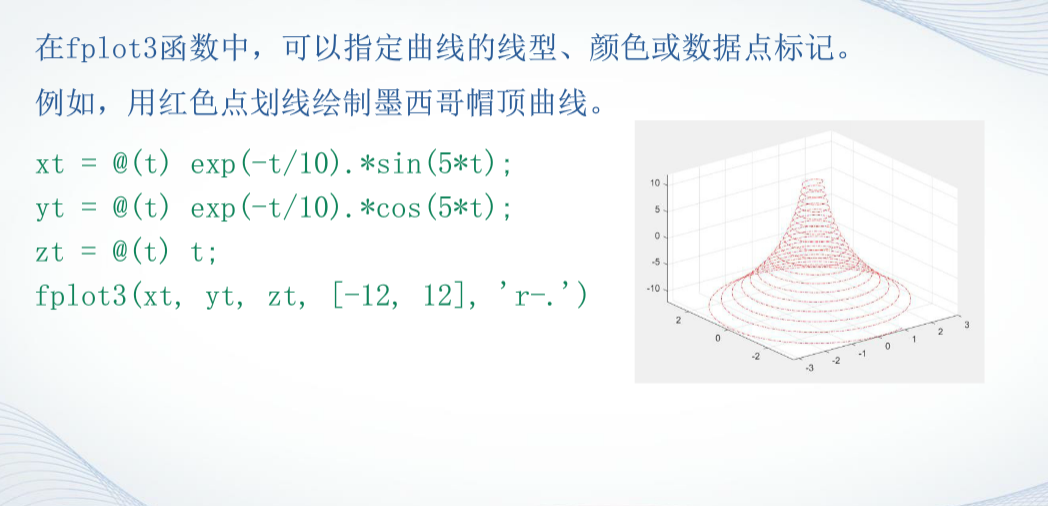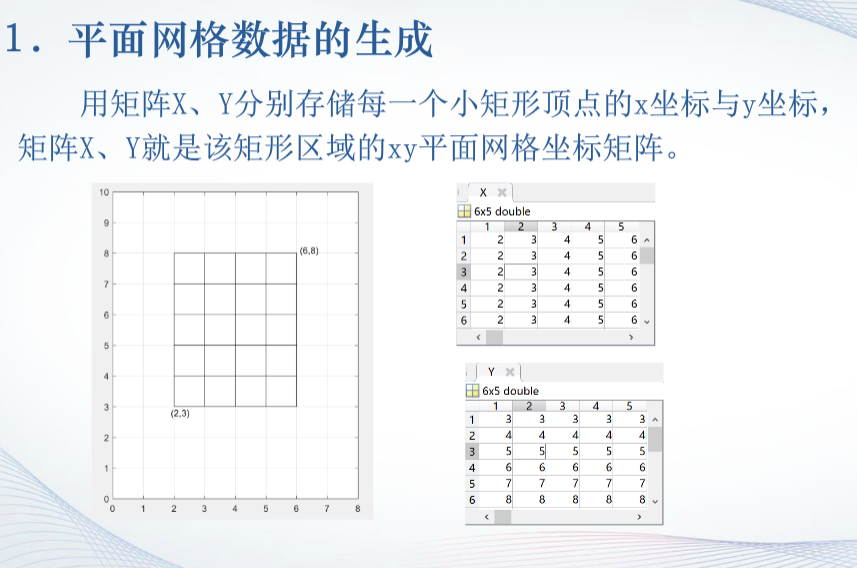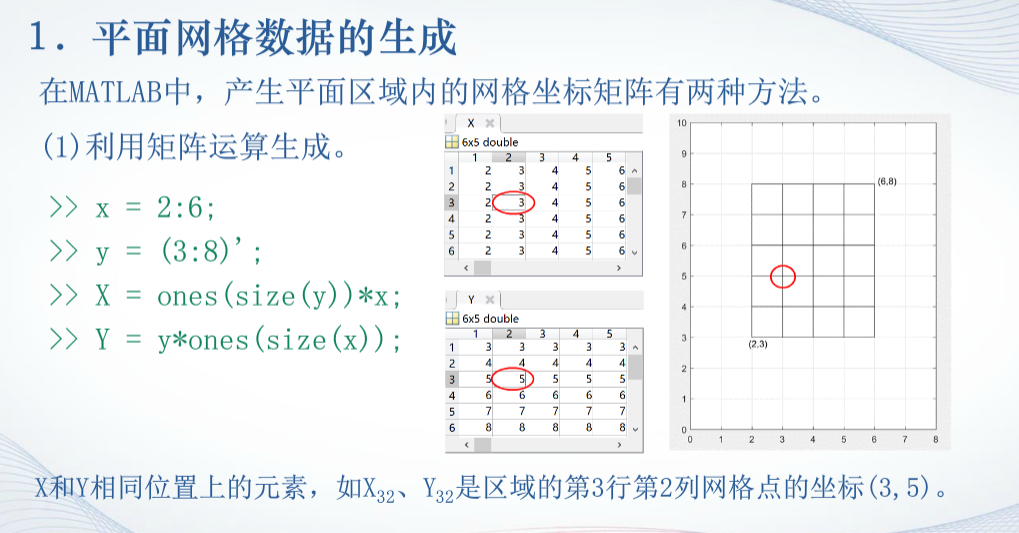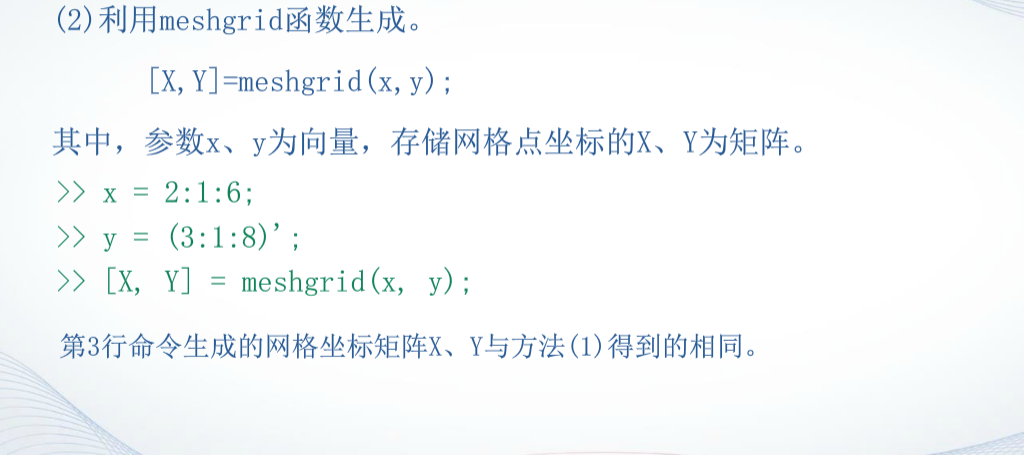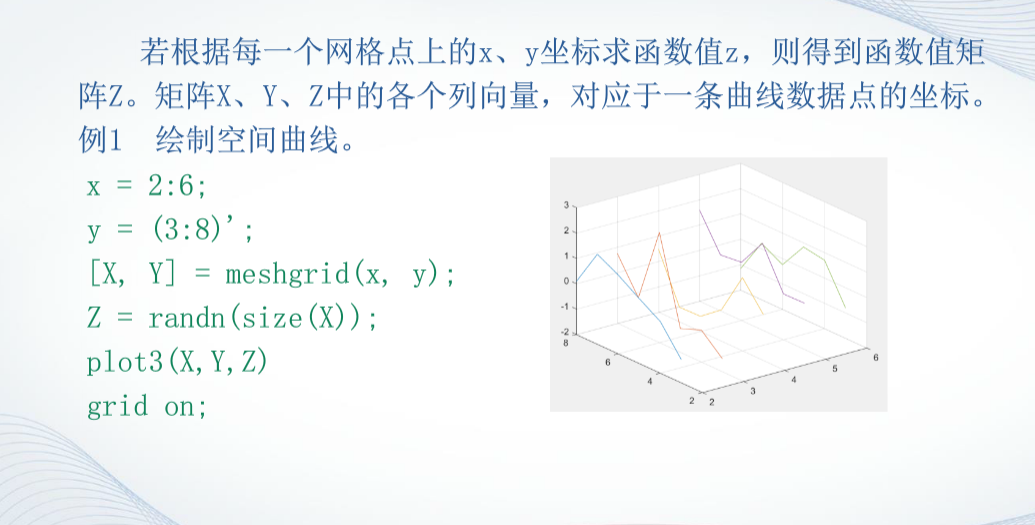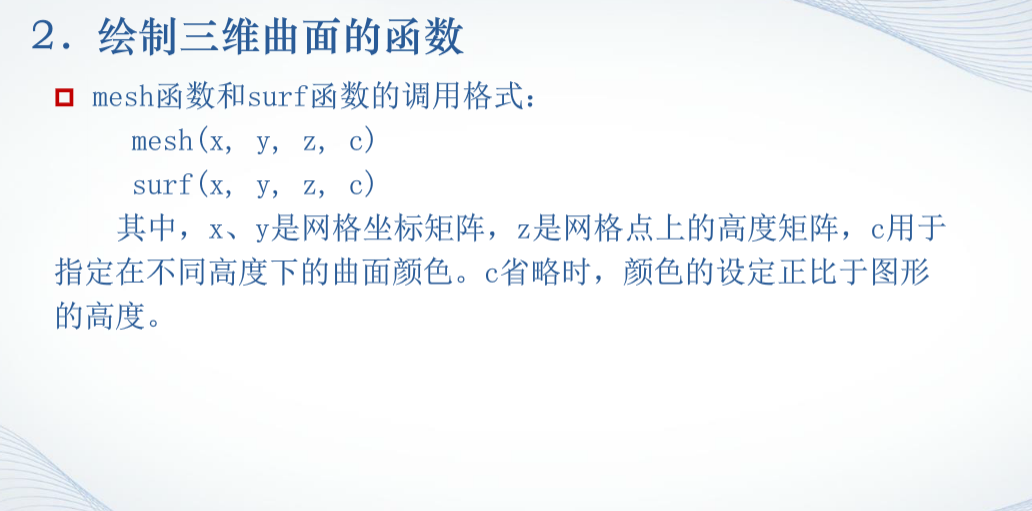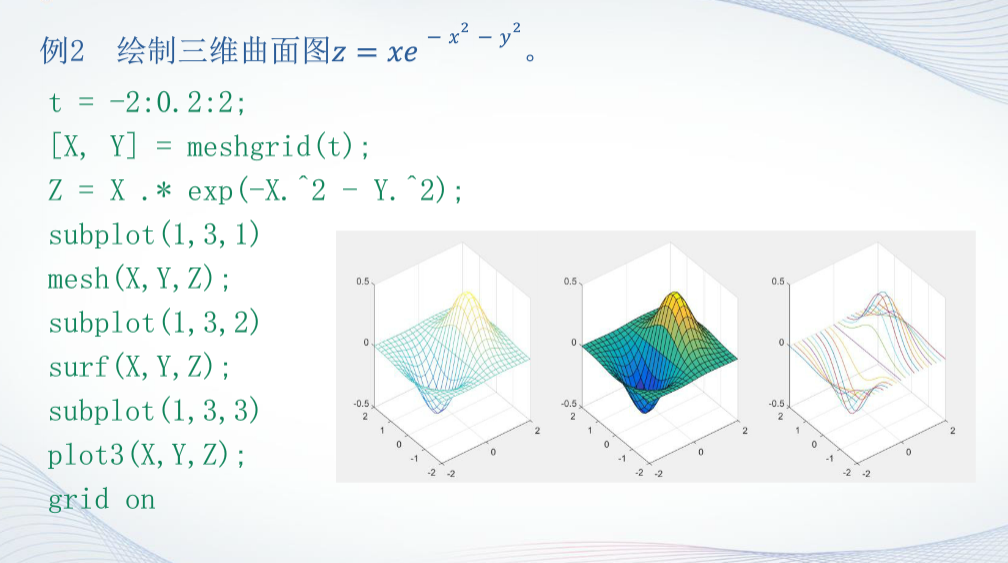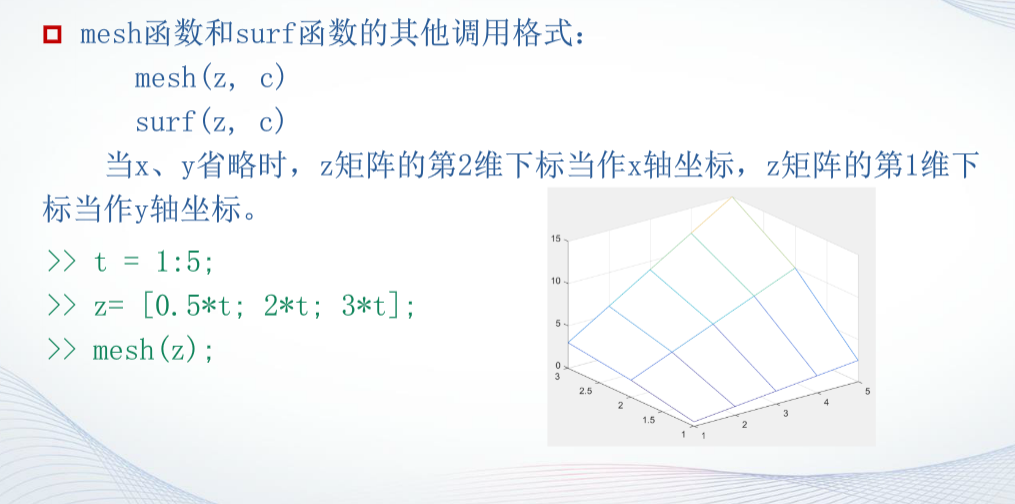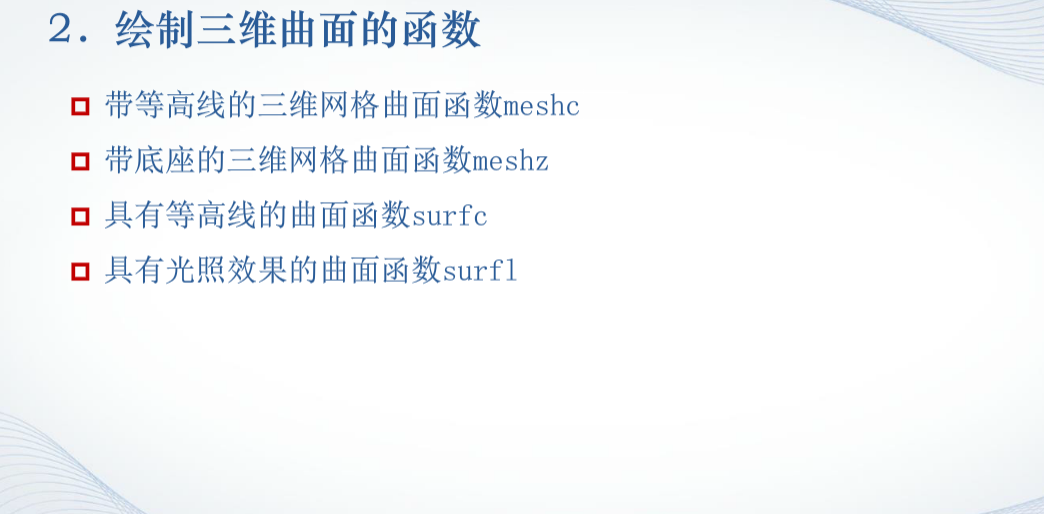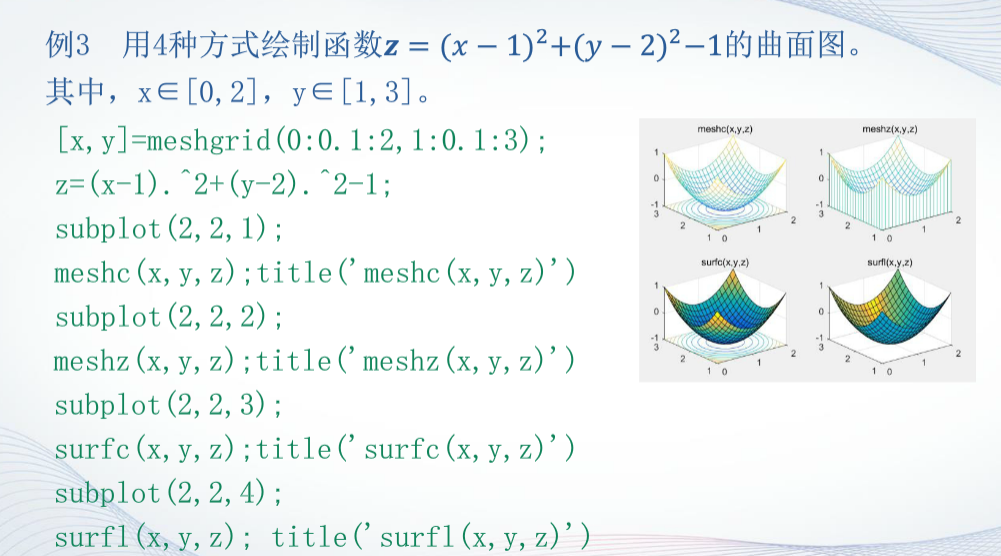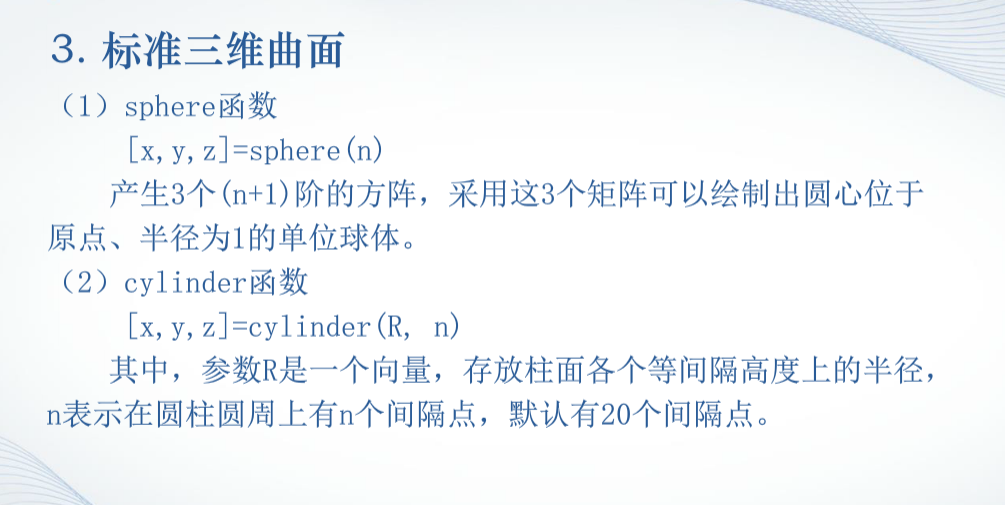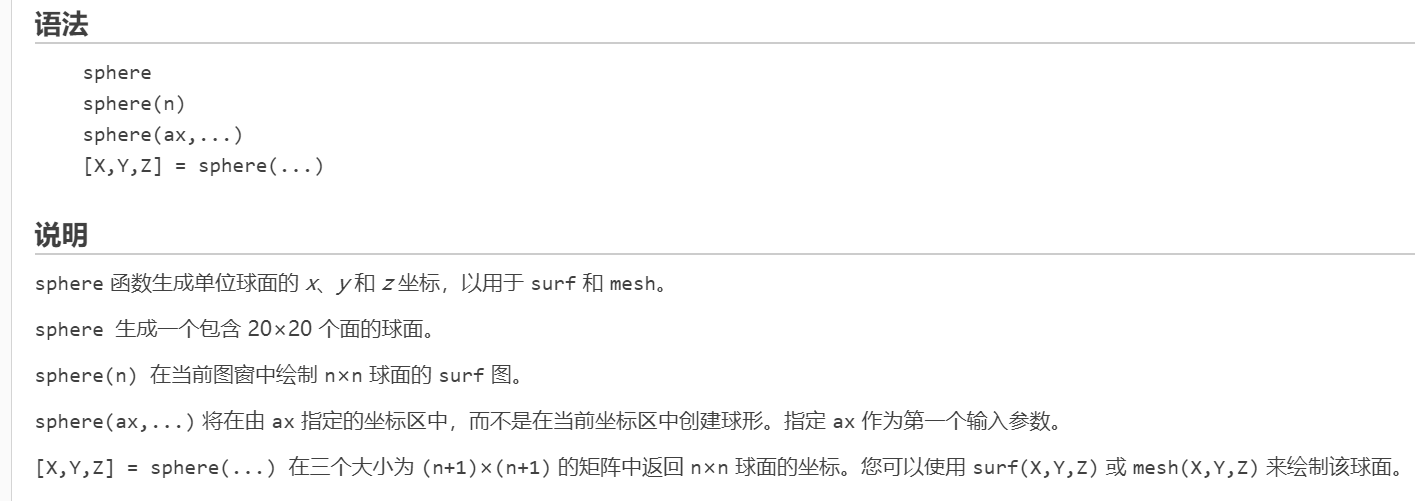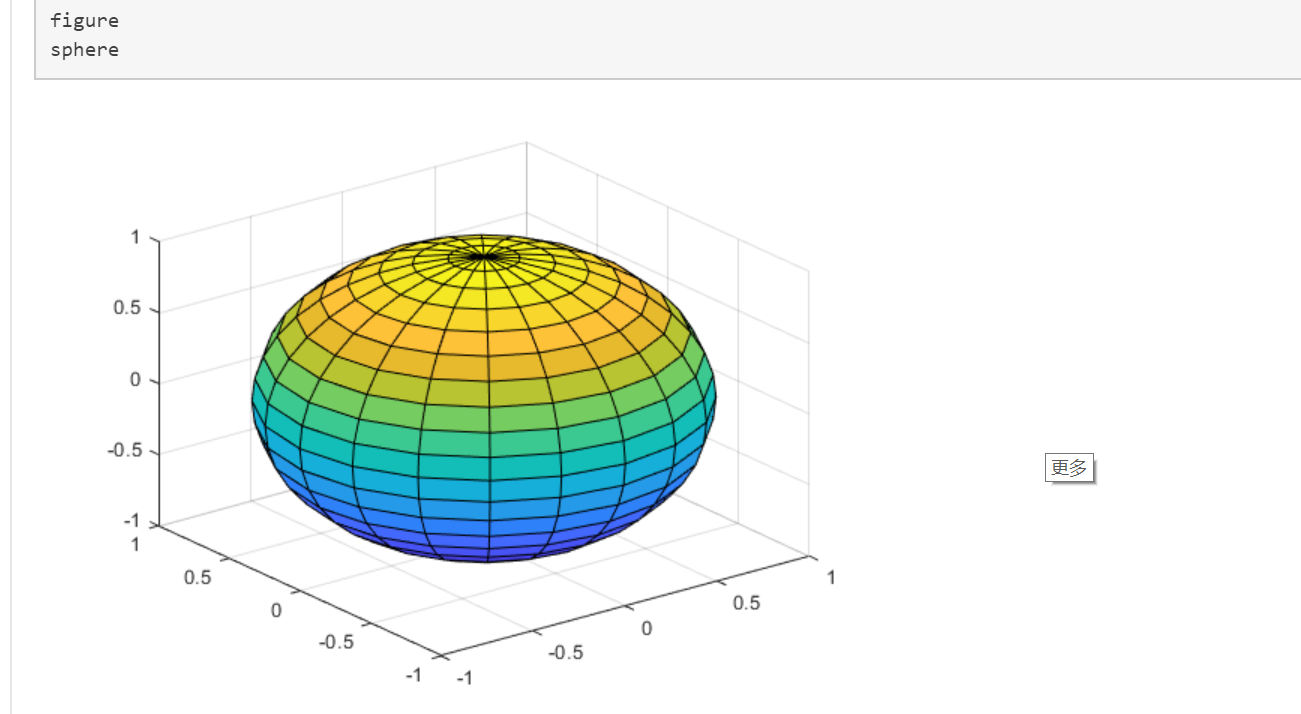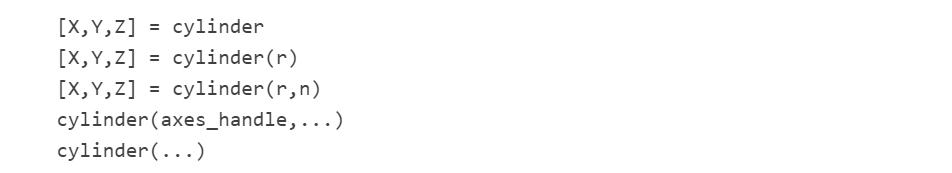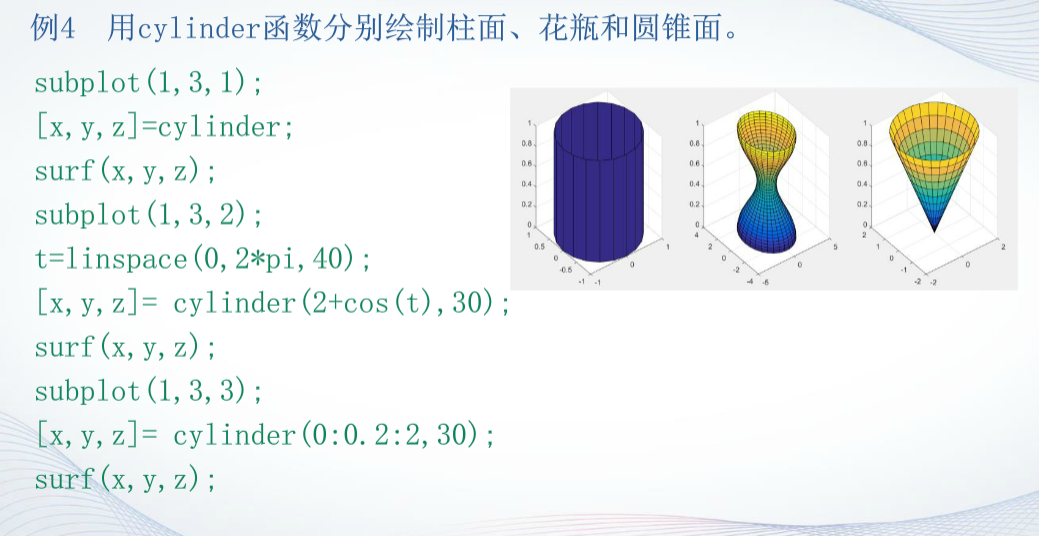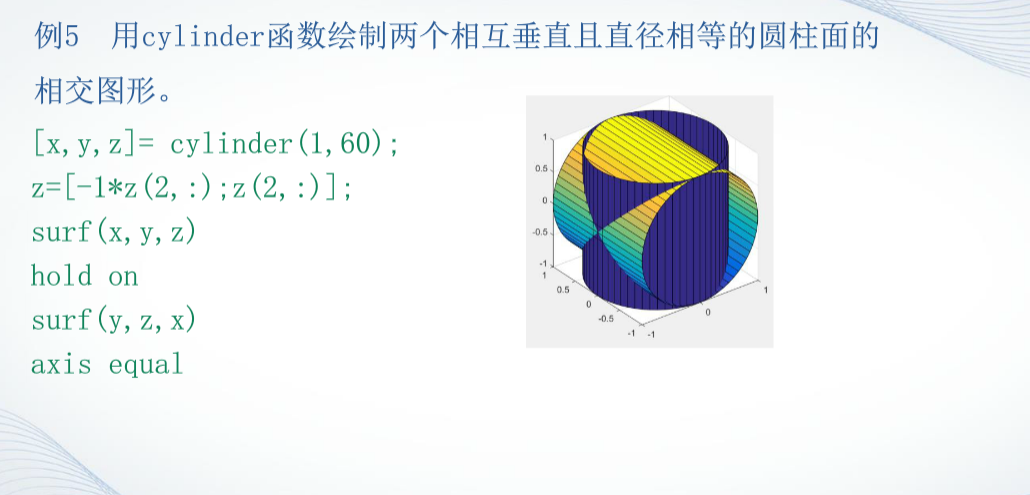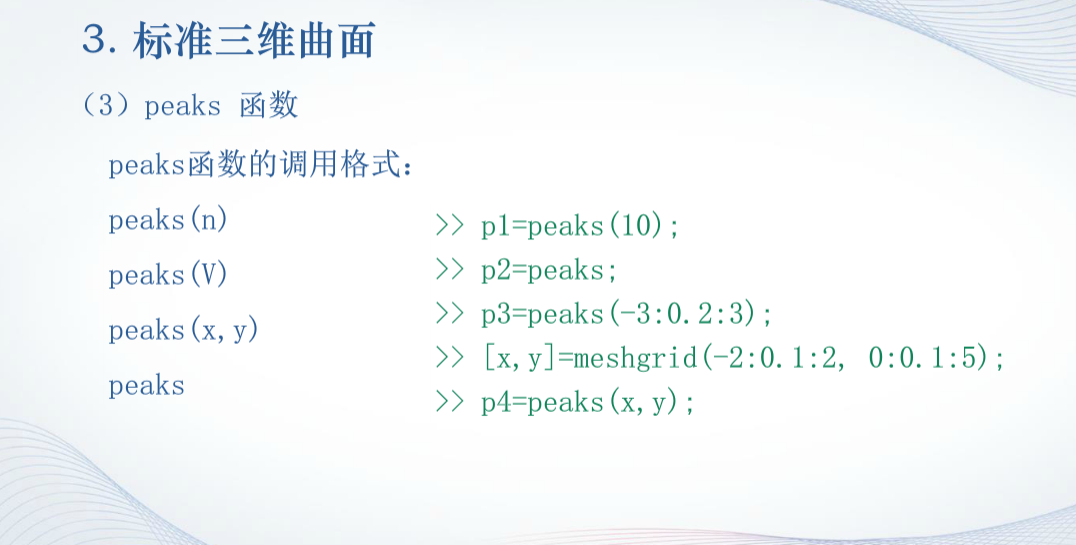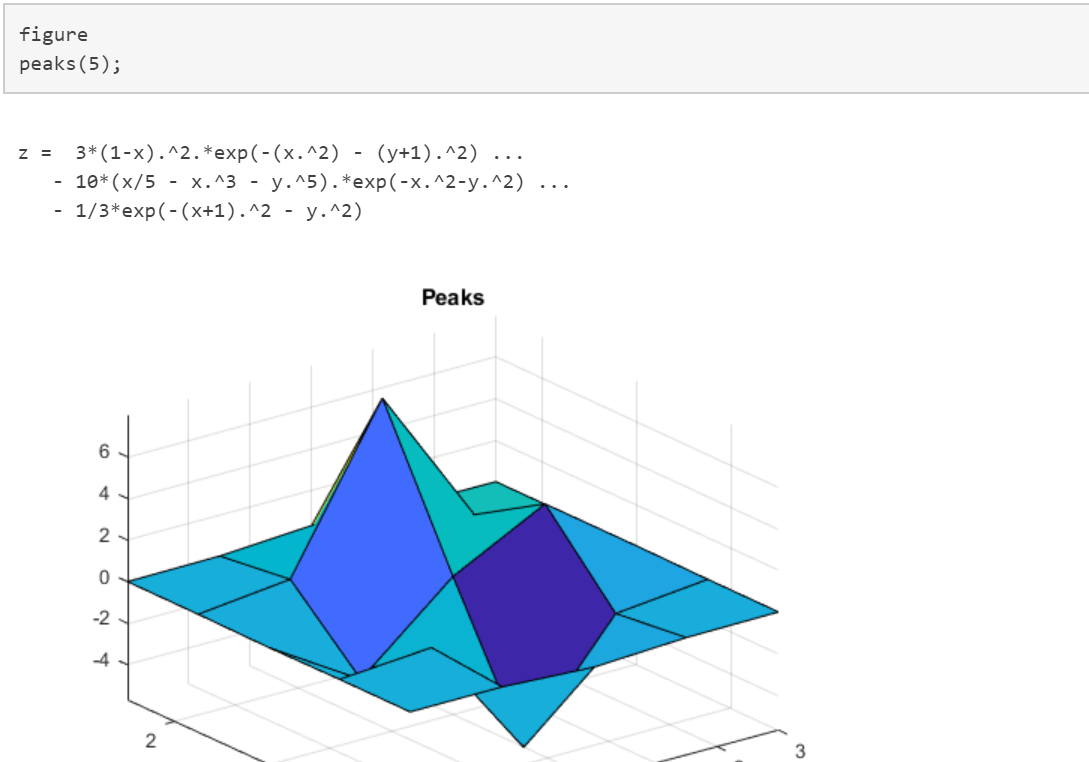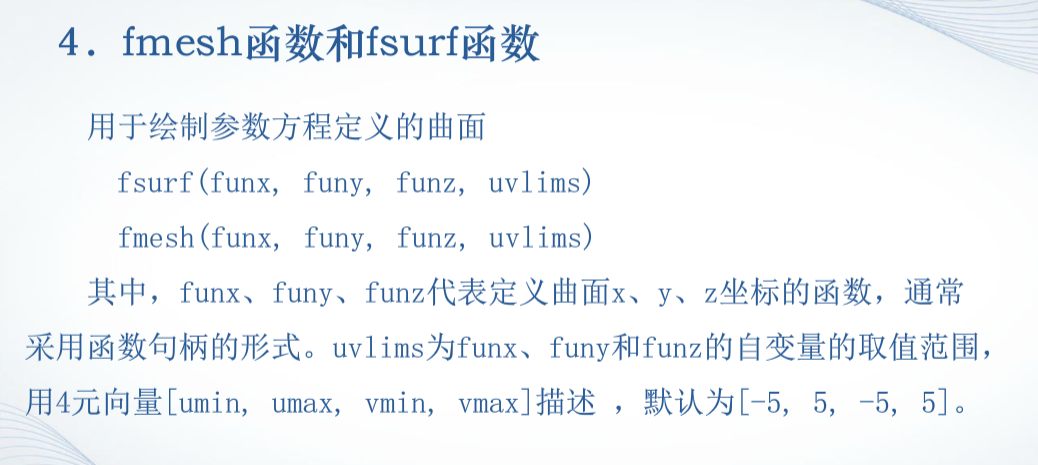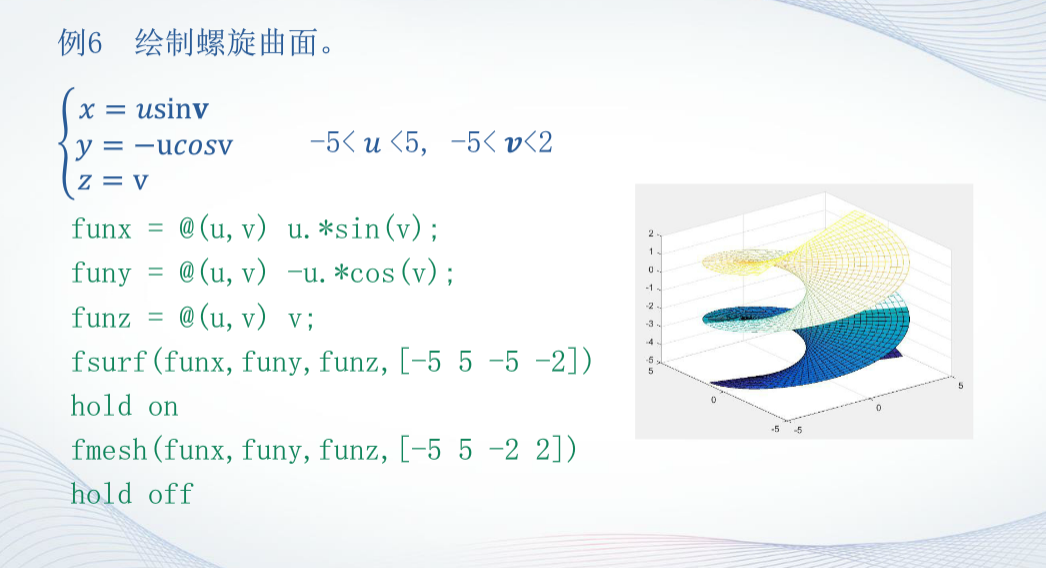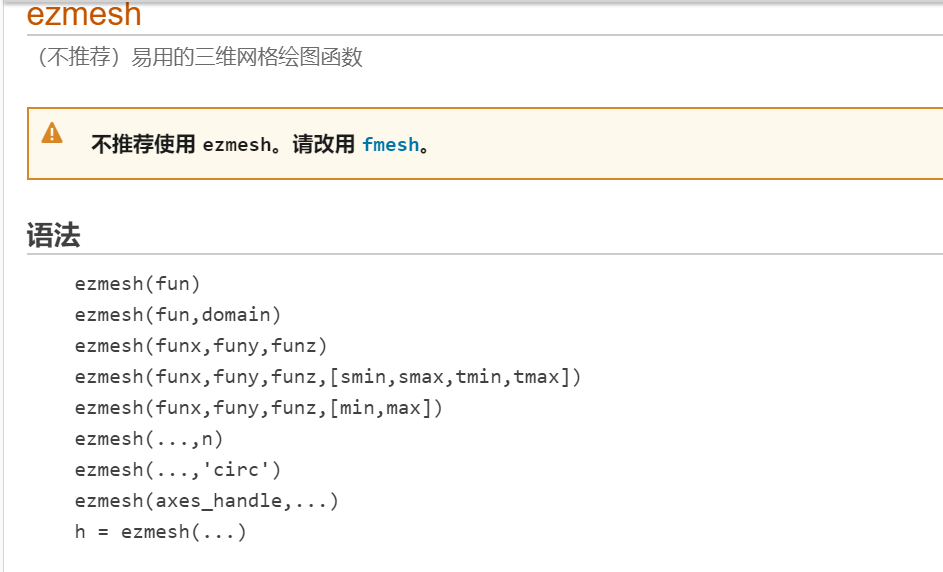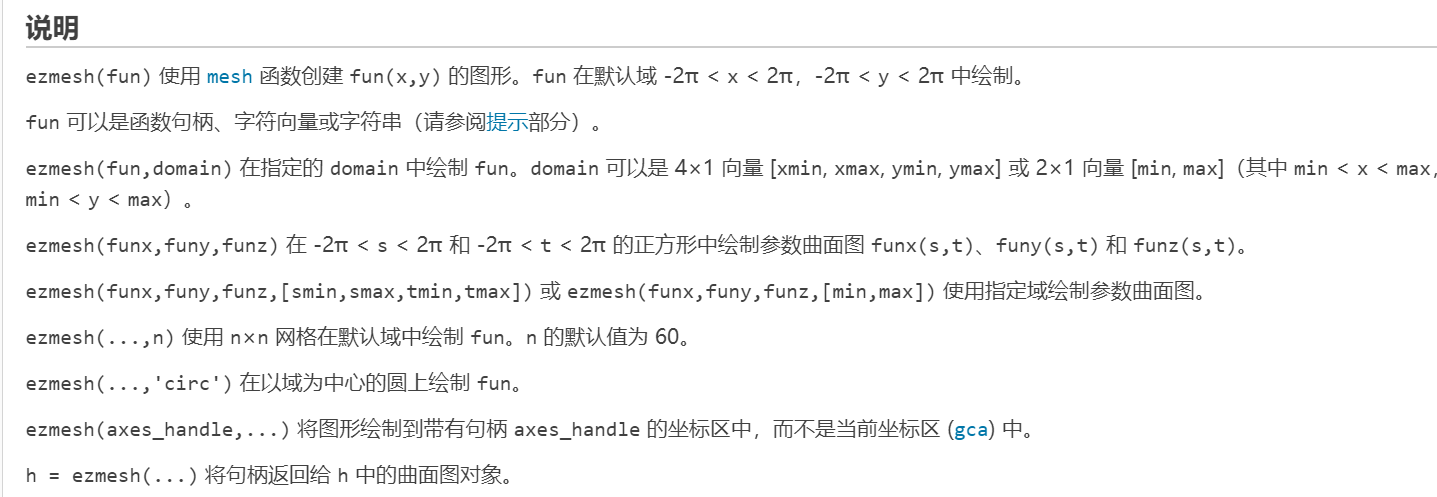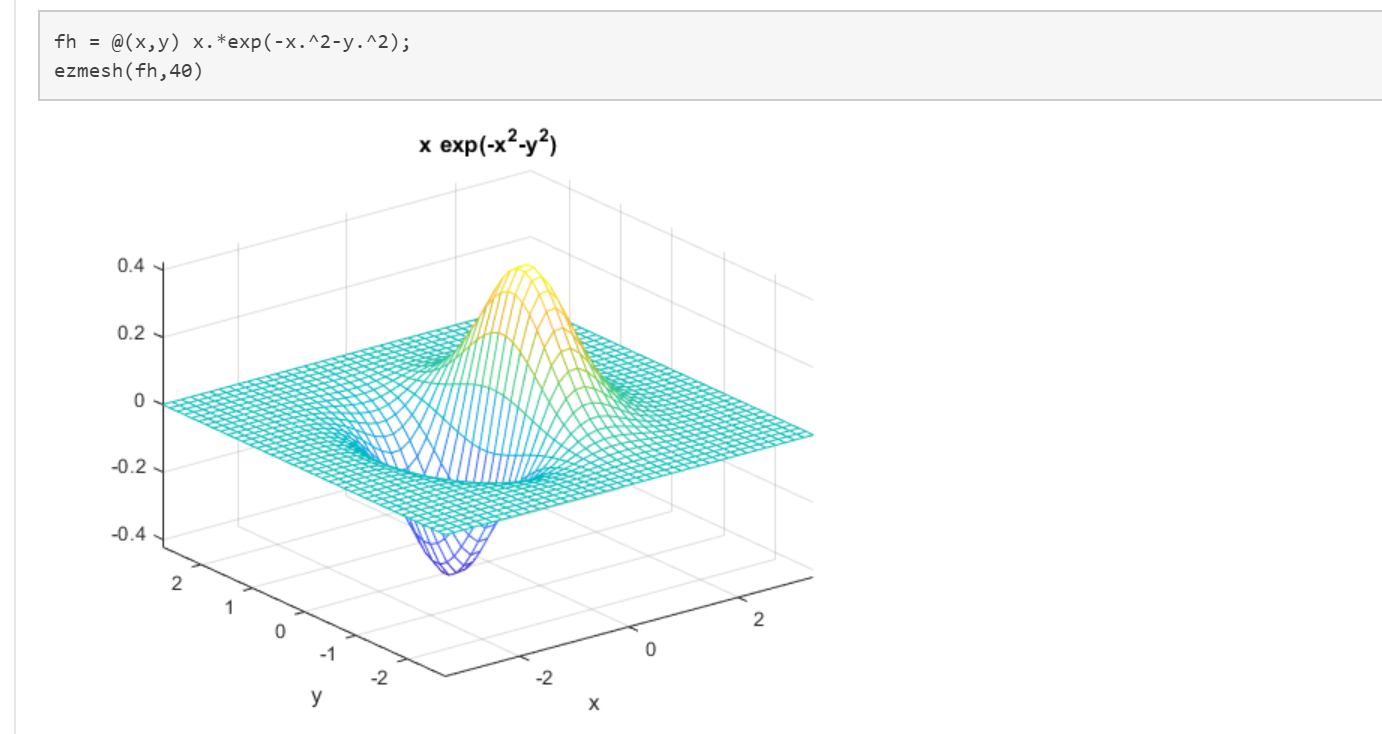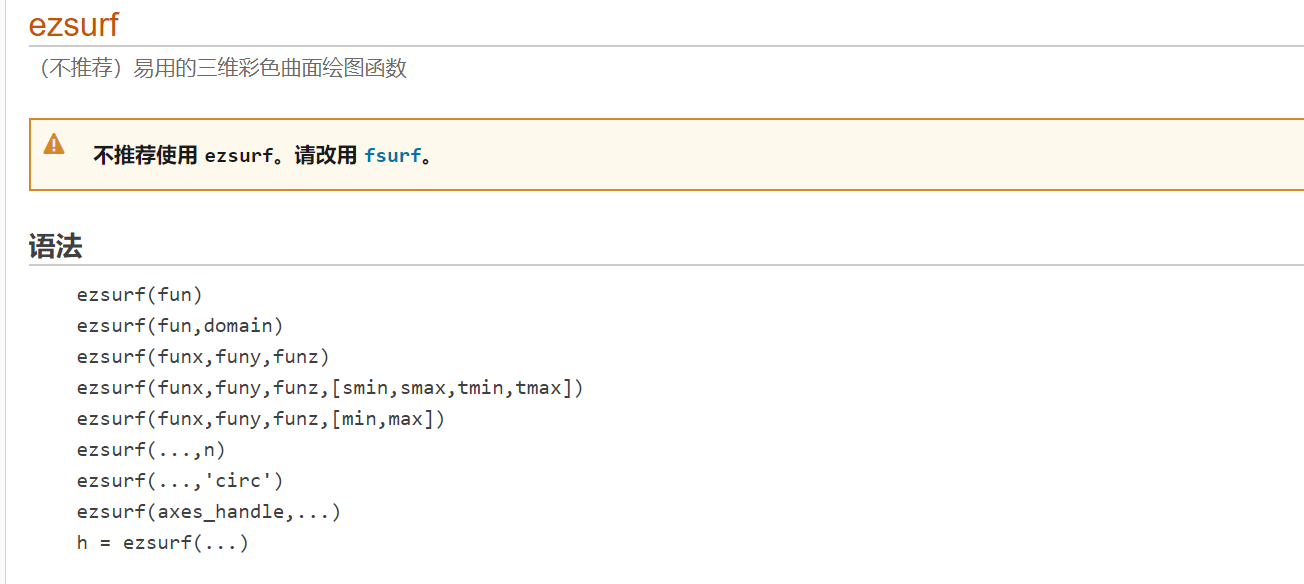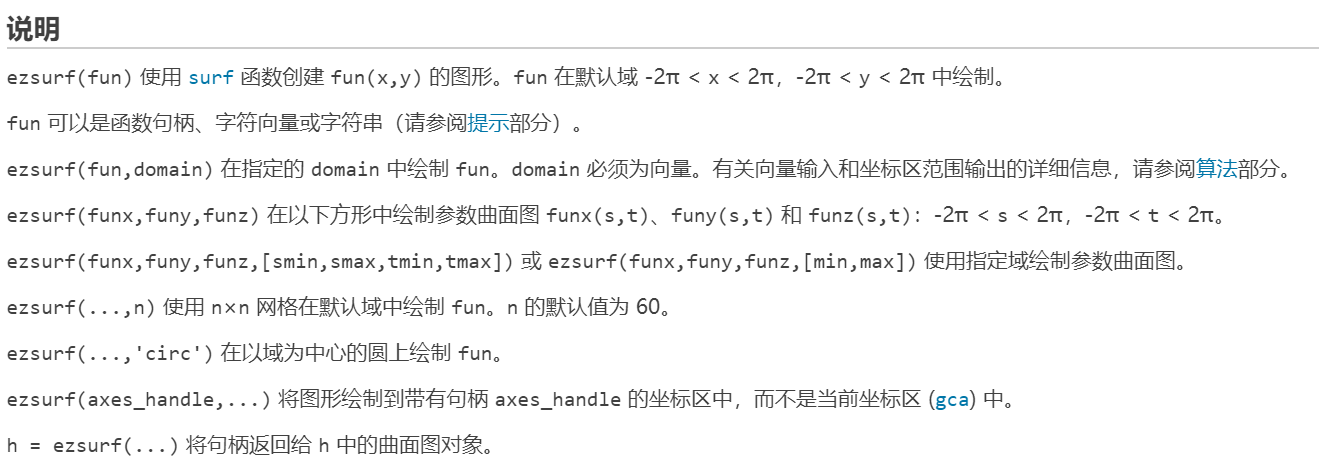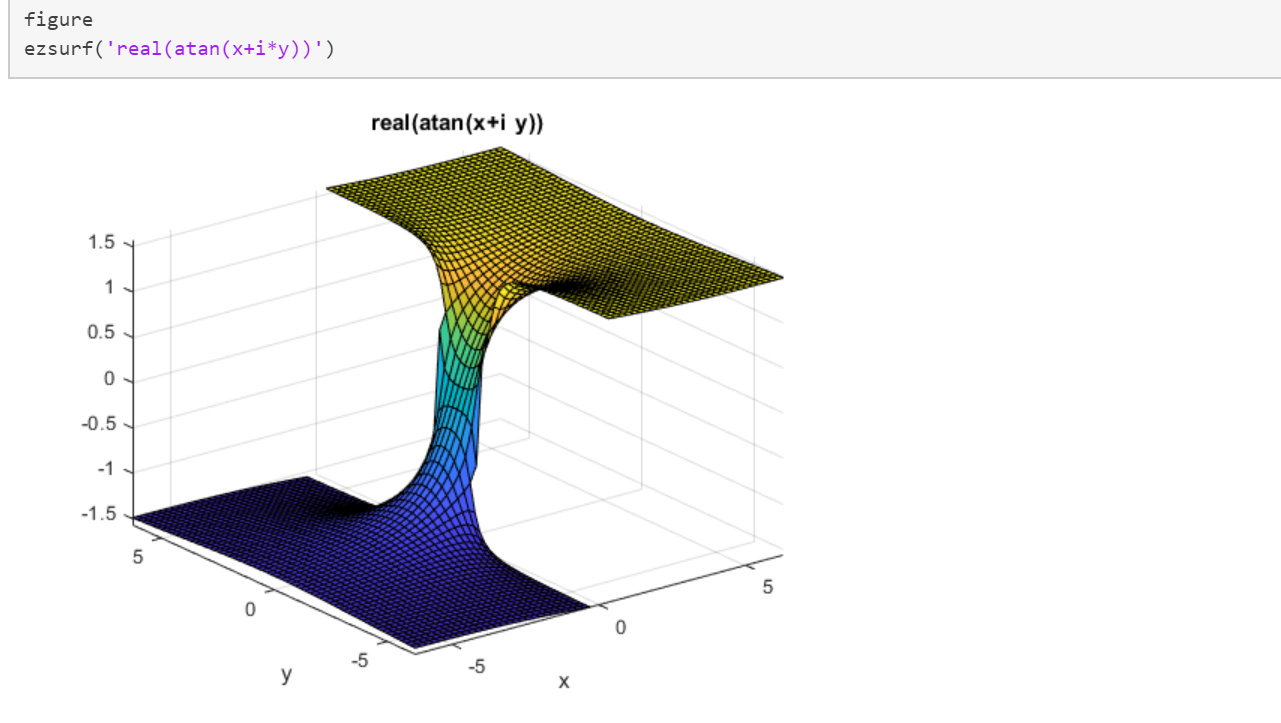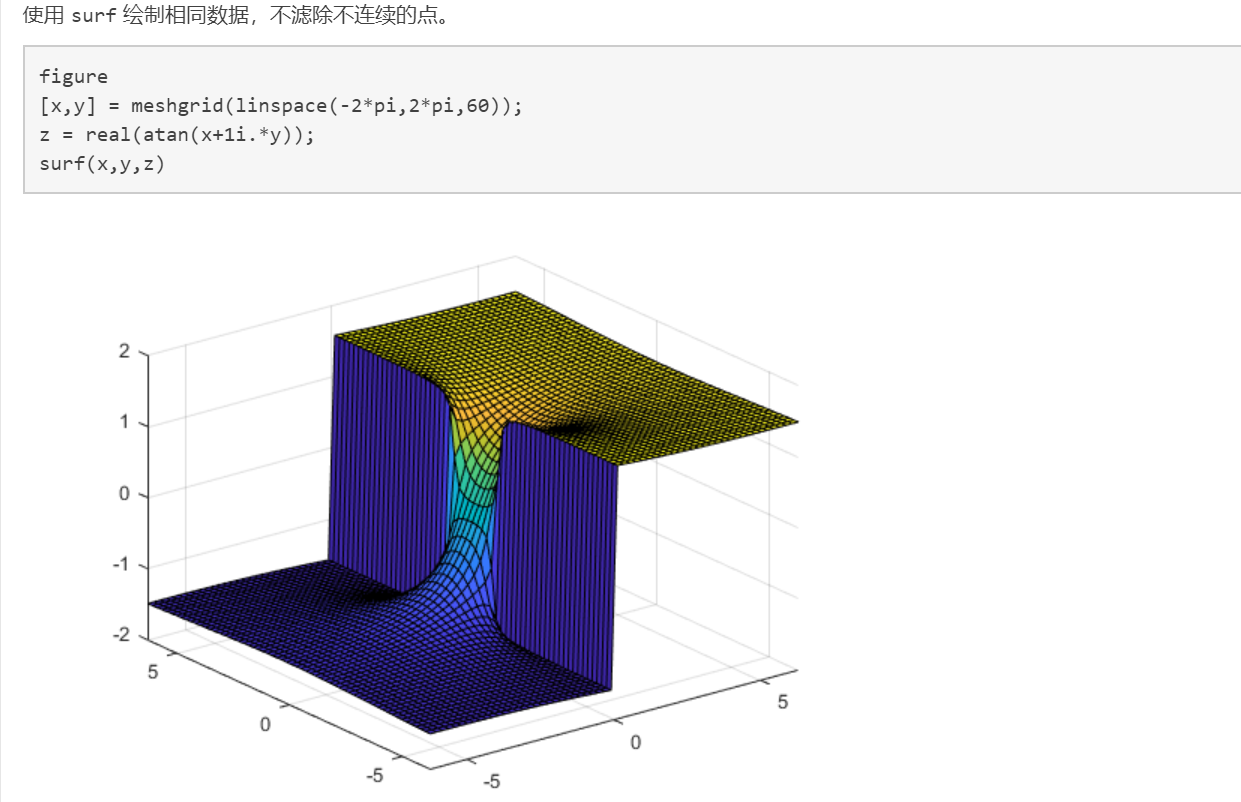展开全文• ## matlab画三维图像

千次阅读 2019-08-22 08:53:45
x = -5:0.01:15; y = 4:0.01:6; [x1, y1] = meshgrid(x, y); z = 21.5 + x1.*sin(4*pi*x1) + y1.*sin(20*pi*y1); plot3(x1, y1, z) x = 11:0.001:12; y = 5.5:0.001:5.8; [x1, y1] = meshgrid(x, y...z = 21.5 + x...
x = -5:0.01:15;
y = 4:0.01:6;
[x1, y1] = meshgrid(x, y);
z = 21.5 + x1.*sin(4*pi*x1) + y1.*sin(20*pi*y1);
plot3(x1, y1, z)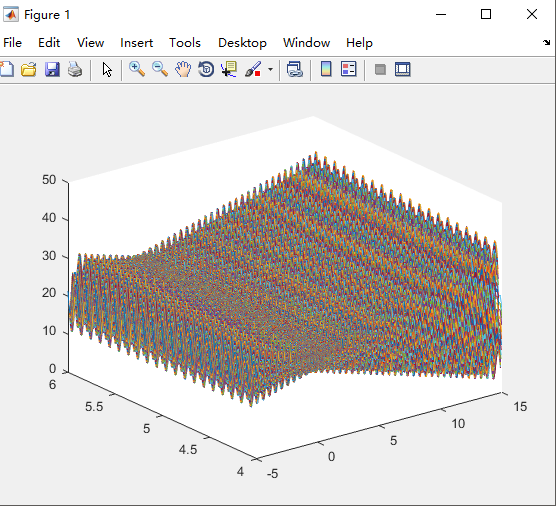x = 11:0.001:12;
y = 5.5:0.001:5.8;
[x1, y1] = meshgrid(x, y);
z = 21.5 + x1.*sin(4*pi*x1) + y1.*sin(20*pi*y1);
mesh(x1, y1, z)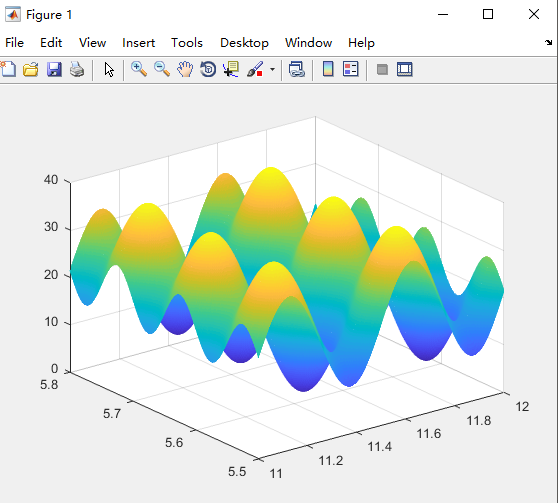展开全文• 主要介绍了matlab画三维图像的示例代码(附demo),文中通过示例代码介绍的非常详细，对大家的学习或者工作具有一定的参考学习价值，需要的朋友们下面随着小编来一起学习学习吧
• 求助 用MATLAB画下边的函数怎么编写代码啊 除x、y、z其余全部已知 ![图片说明](https://img-ask.csdn.net/upload/201906/06/1559787937_625012.png)r语言 开发语言
• ## matlab画三维图像（plot3,mesh,surf）

万次阅读 多人点赞 2019-06-12 10:26:41
二维函数的图像必须用三维坐标系，很多函数的图像长得很好看，很有意思。会可视化也会有利于我们理解更深刻。 （1）plot3,三维曲线图 在网上看到这图觉得挺好看： t=0:pi/360:2*pi; x=sin(t); y=cos(t); z=2...
二维函数的图像必须用三维坐标系画，很多函数的图像长得很好看，很有意思。会可视化也会有利于我们理解更深刻。
（1）plot3,三维曲线图
在网上看到这图觉得挺好看：
t=0:pi/360:2*pi;
x=sin(t);
y=cos(t);
z=2*x.^2+y.^2;
figure
plot3(x,y,z,'Color','r','LineWidth',2);
xlabel('x');
ylabel('y');
zlabel('z');
title('z=(cos t)^2+2*(sin t)^2');
axis([-1.2 1.2 -1.2 1.2 1 2.2]);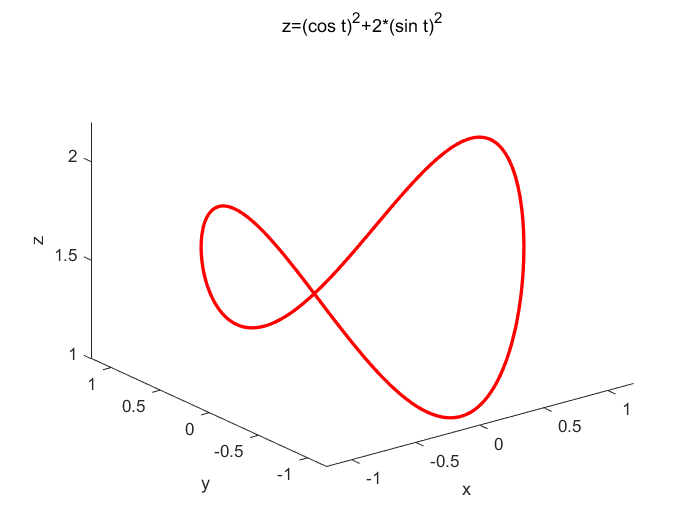（2）mesh,网面图
clear all,clc;
t=-0.5:.01:0.5;
[x,y]=meshgrid(t);%形成格点矩阵
z=sin(4*pi*x)+cos(6*pi*y);
figure(1)
mesh(x,y,z);
axis([-0.5 0.5 -0.5 0.5 -2 2]);
title('z=sin(4*pi*x)+cos(6*pi*y); mesh')
colormap cool%cool是一种配色方案，还有其他方案如winter，summer····见help colormap
colorbar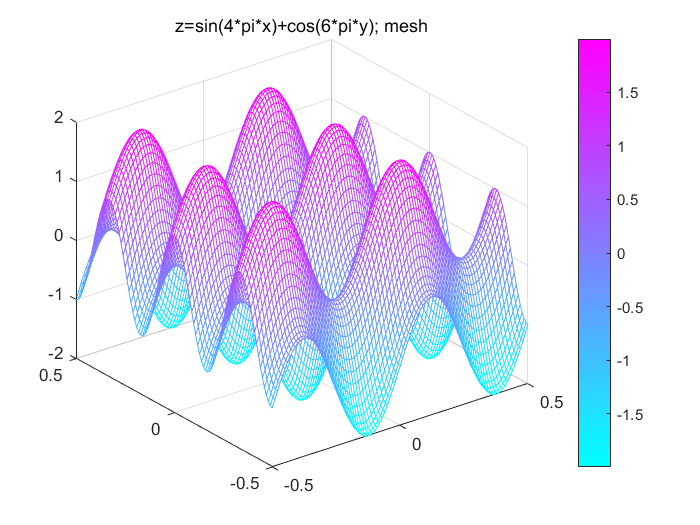画个二元函数，观察采样后频谱的混叠现象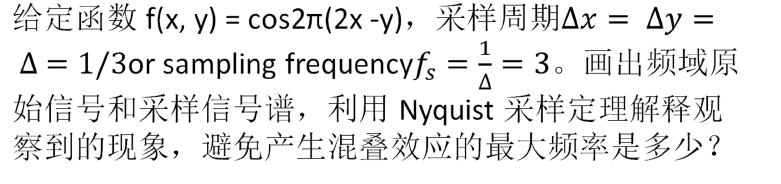二元函数图像的绘制还是把变量分为多个离散点绘制，只是增大点数。
clear all,clc;
t=-1:.01:1;
[x,y]=meshgrid(t);%形成格点矩阵
f=cos(2*pi*(2*x-y));
figure(1)
mesh(x,y,f);grid on
xlabel('x');
ylabel('y');
zlabel('f');
axis([-0.5 0.5 -1 1 -1.5 1.5]);
title('f=cos[2*pi(2x-y)]; mesh')
colormap winter
colorbar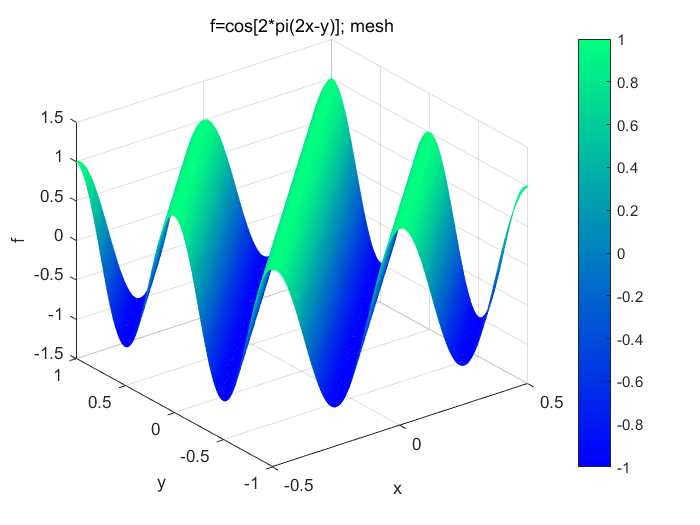先手动计算一下它的二位傅里叶变换：
不想打公式（逃）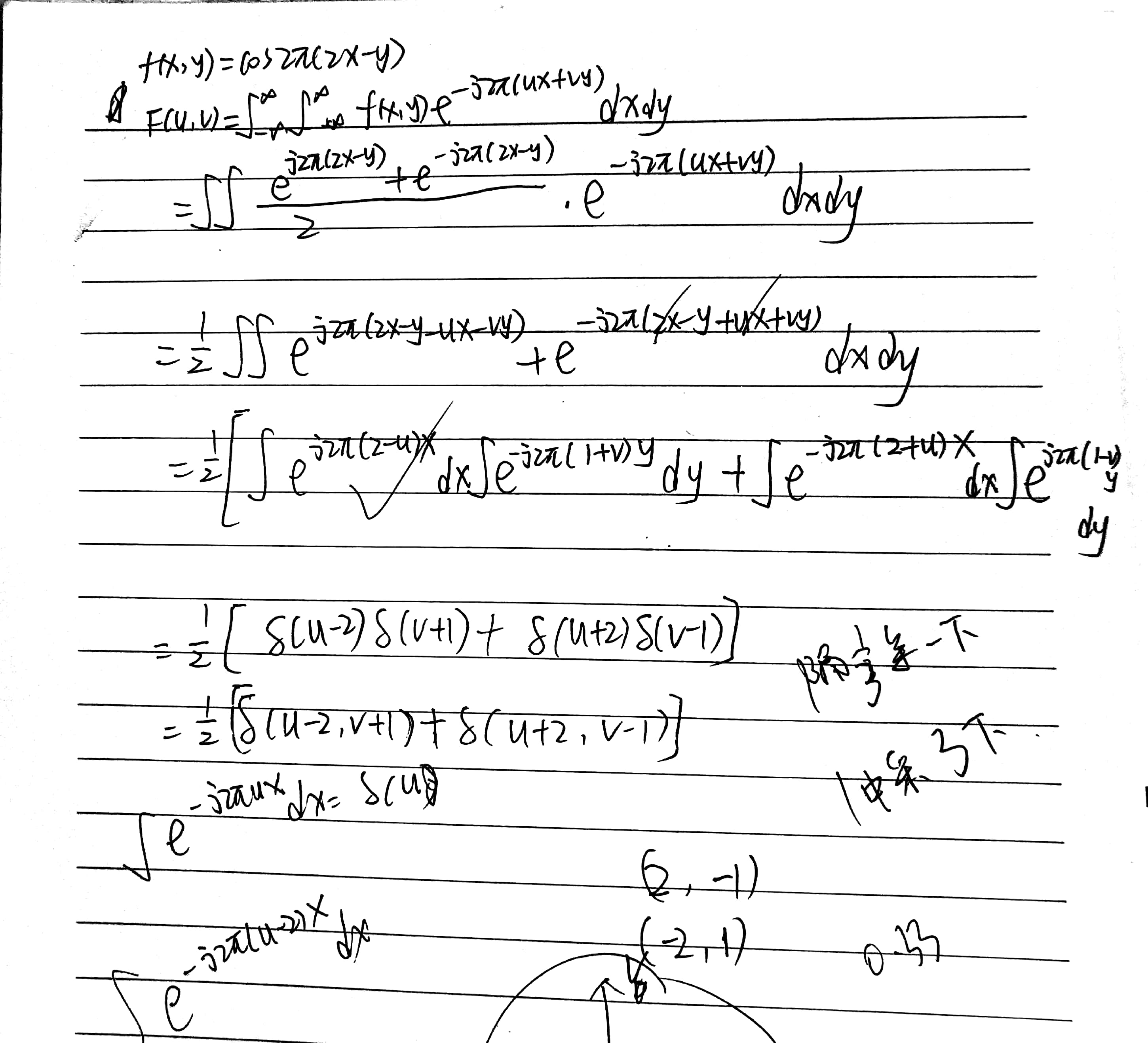F= fft2(f);%做二维DFT变换，把连续函数取样得到一个矩阵，把这个矩阵当图像一样处理
figure(2)
subplot(121),imshow(abs(F),[]);title('函数f=cos(2*pi(2x-y))二维频谱')
F=fftshift(F);%使用matlab的fftshift函数把低频分量移到频谱中心
subplot(122),imshow(abs(F),[]);title('中心化频谱')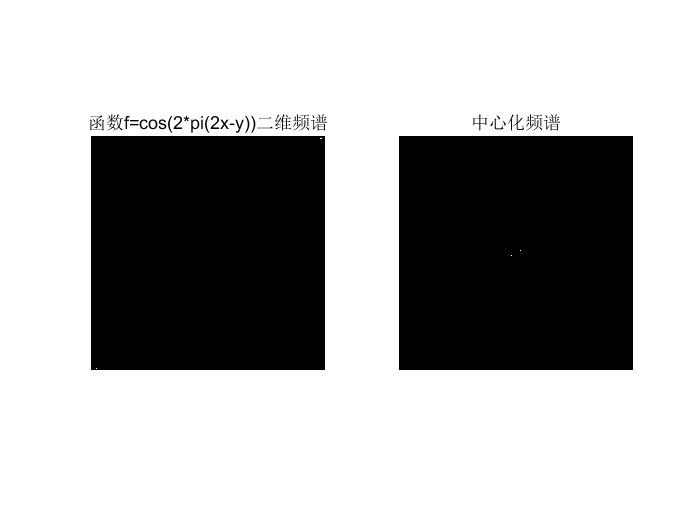t=-1.1:.333:1.1;%以频率3采样（题目要求）
[x,y]=meshgrid(t);%形成格点矩阵
f=cos(2*pi*(2*x-y));
figure(1)
mesh(x,y,f);grid on
xlabel('x');
ylabel('y');
zlabel('f');
axis([-1 1 -1 1 -1.5 1.5]);
title('采样后的f=cos[2*pi(2x-y)]; mesh')
colormap winter
colorbar

F= fft2(f);%做二维DFT变换，把连续函数取样得到一个矩阵，把这个矩阵当图像一样处理
figure(2)
subplot(121),imshow(abs(F),[]);title('函数f=cos(2*pi(2x-y))采样后的二维频谱')
F=fftshift(F);%使用matlab的fftshift函数把低频分量移到频谱中心
subplot(122),imshow(abs(F),[]);title('中心化频谱')

采样后信号：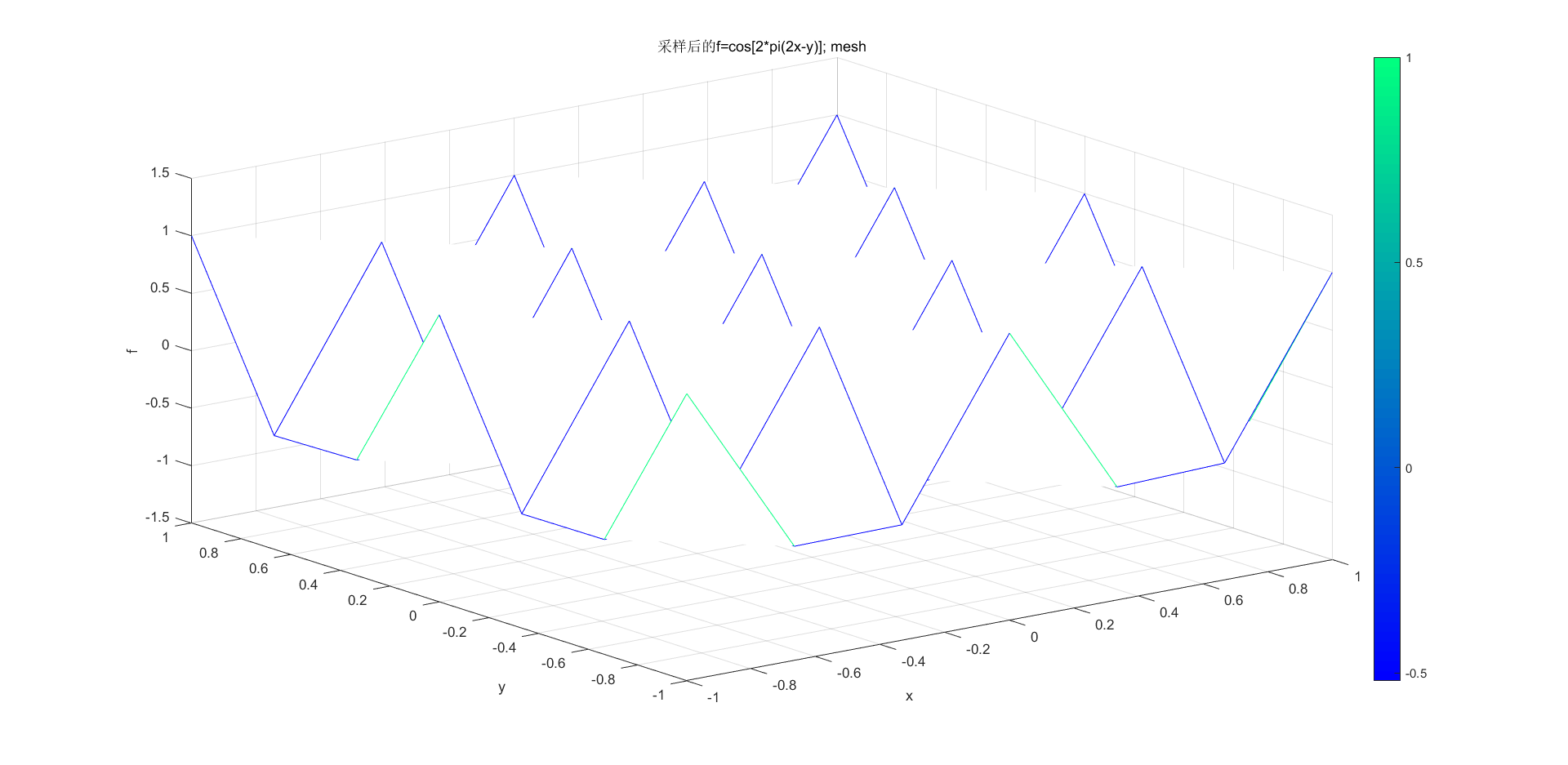采样后信号频谱：
函数的x方向频率是2，y方向频率是1，所以一定会有混叠失真
可以看出频谱的能量中心的相对位置都不对，这就是混叠失真引起的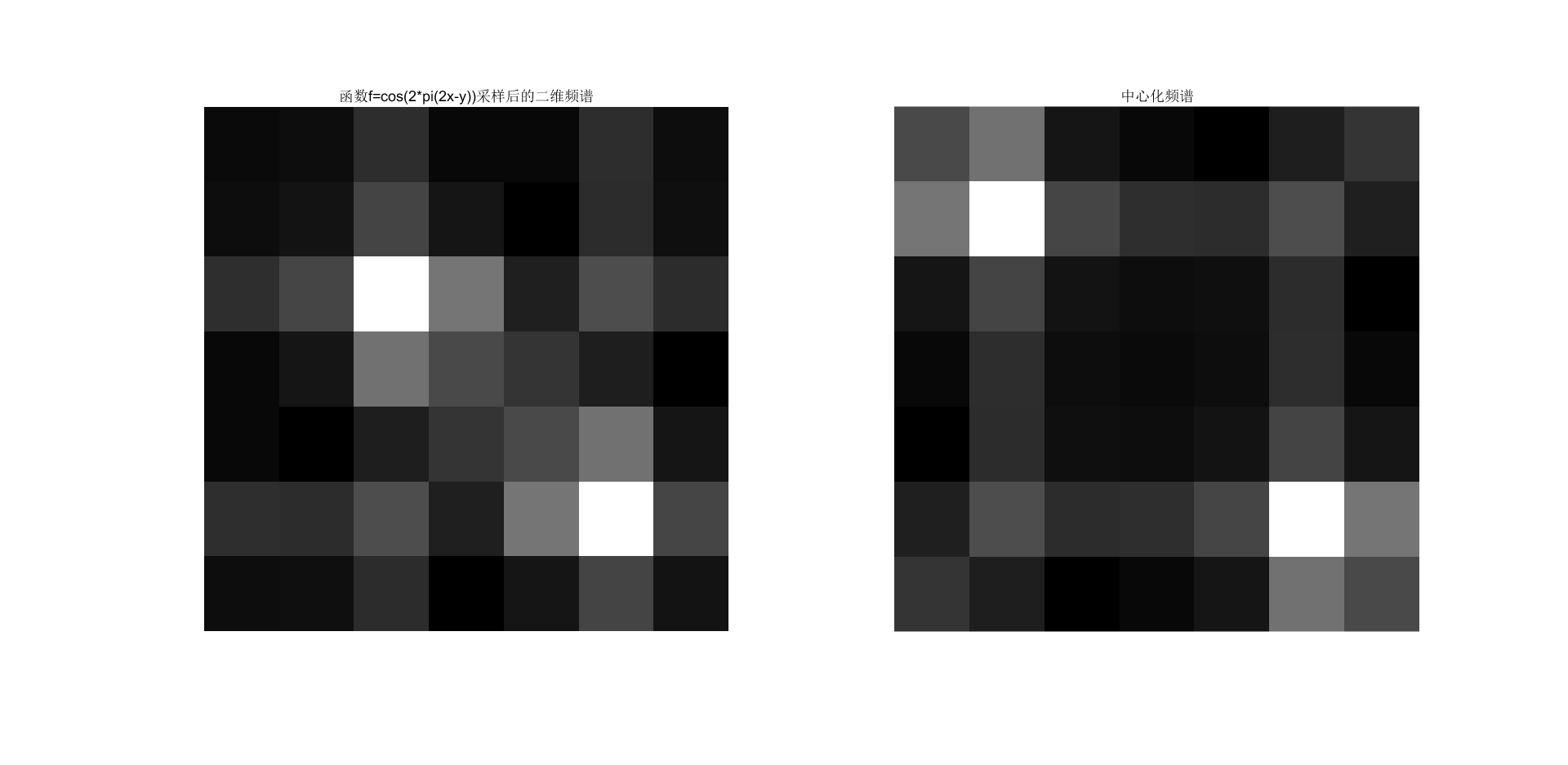t=-1.1:.17:1.1;%以更高频率采样
[x,y]=meshgrid(t);%形成格点矩阵
f=cos(2*pi*(2*x-y));
figure(1)
mesh(x,y,f);grid on
xlabel('x');
ylabel('y');
zlabel('f');
axis([-1 1 -1 1 -1.5 1.5]);
title('采样后的f=cos[2*pi(2x-y)]; mesh')
colormap winter
colorbar

F= fft2(f);%做二维DFT变换，把连续函数取样得到一个矩阵，把这个矩阵当图像一样处理
figure(2)
subplot(121),imshow(abs(F),[]);title('函数f=cos(2*pi(2x-y))采样后的二维频谱')
F=fftshift(F);%使用matlab的fftshift函数把低频分量移到频谱中心
subplot(122),imshow(abs(F),[]);title('中心化频谱')

以更大的采样频率采样，避免不满足奈奎斯特定理产生混叠：
可见得到的频谱和连续函数的频谱更相似。采样频率越大，得到的频谱和连续函数的频谱越相似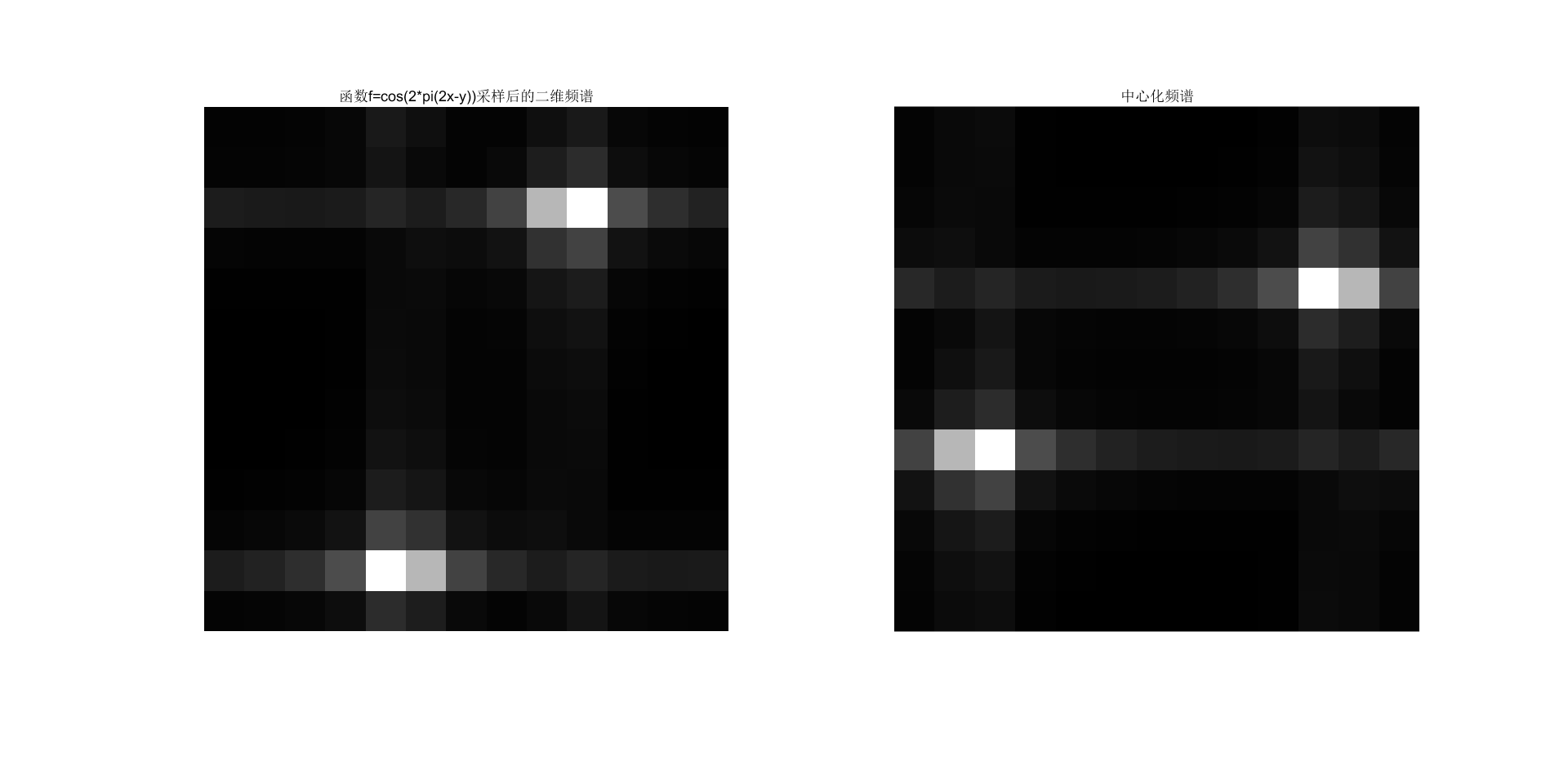展开全文• clear;clc close all; load seamount;% 求data的最大最小值 minX = min(x); maxX = max(x);...% 出一平面 [x1 , y1] = meshgrid(minX:0.01:maxX, minY:0.01:maxY);% 散乱点插值 z1 = griddata(x, y, z,
• x=xlsread(‘excel 文件位置’，第一行)； y=xlsread(‘excel 文件位置’，第一列)； z=xlsread(‘excel 文件位置’，行和列对应内容)； [x1,y1]=meshgrid(x,y); figure mesh(x1,y1,z)
• 绘画出图像灰度值的三维图像 方法一： i=imread('a1.jpg'); if(size(i,3)>1) i=rgb2gray(i); end i=double(i); mesh(i); figure,mesh(flipdim(i,1)),title('水平镜像翻转后 这样更加符合MATLAB对于图像处理...数字图像处理
• 直接用mesh函数可输出三维图，例子来自带有偏移量的两幅图片用相位相关法做的位移量计算 Image1 = (imread('image1.jpg')); Image2 = (imread('image2.jpg')); // % 带有偏移量的图像 Image1=X1; Image2=X2; // %...
• MATLAB画三维球体、半球体、圆柱、山峰等图像，源代码，可直接运行
• 提问：按照讲课的PPT中的数学公式做三维图，运行出来的图为什么跟老师的完全不一样? 解答：公式输入不规范 更改后代码： [X,Y]=meshgrid(0:0.001*pi:1); Z=(3.9*exp((-0.25)*(9*X-2).^2-0.25*(9*Y-2).^2)+3.9*exp(...
• 这是本系列经验的第八篇，主要介绍用matlab软件画三维图像的一些相关函数，使用这些函数可以很方便的画出想要的图形，非常直观好用。 网状图:  x=linspace(-2, 2, 25); % 在x轴...
• 如果你学习过matlab，对一些三维图像显示，感觉很吃惊，数据还能这么美丽地显示出来，其实python里使用numpy也同样显示这么美丽的图像出来的。如下例子：#python 3.6 #蔡军生 #...python tensorflow numpy
• 基于Matlab软件编程实现MC算法对人体脑部MRI图像进行面绘制三维重建；利用三维旋转变换和动画函数对脑部图形进行动画显示。实验结果表明，该算法能准确地对脑部进行全方位显示，运行速度快且占的内存空间较少。
• 二元函数，即两个变量可以得到三维图像，下面通过一个例子进行讲解。 首先利用meshgrid函数产生平面区域内的网格坐标矩阵。 x = [1,2,3,4,5] y = [2,4,6]; [X,Y]=meshgrid(x,y); 执行完以后X、Y均为矩阵，...
• 最近要用到matlab 来进行可视化显示以下数据，把数据导入matlab 之后 是二数组的数据，想以数组的x和y 分别为x 和y 轴，然后以数组相对应的数据为z 轴，然后进行画图显示clear ;clc; Z=importdata('D:\chy\z\1.txt...
• 为了便于观察图像的像素值分布，我们常常需要将灰度图像化成三维图，观察起来更加直观。 今日发现，直接用mesh（你的图）就可以将二维矩阵三维图，然而网上大部分教程都是巨坑，非得教人生成网格再用meshgrid...
• 为了便于观察图像的像素值分布，我们常常需要将灰度图像化成三维图，观察起来更加直观。mesh（）出来的图可能发生垂直镜像，只要再镜像一次就好了，mesh（flipdim（你的图，1））。
• matlab求二维标量场的梯度，并出该标量场的三维图像及其梯度场图像。文件夹包括一份仿真结果，以及用matlab进行求梯度、求三维图像、求梯度图这三次的程序和程序截图，还有一份标量场的三维图像，一份标量场梯度...
• Matlab压缩后画三维图代码低聚 CSCI 4830：计算机视觉最终项目（Prayash Thapa和Martin Fejka） 最初的想法 我们的想法是创建一个iOS应用程序，该应用程序将从用户拍摄的图片中生成艺术图像。 这些照片可以实时拍摄...
• 现有一个400*400二维矩阵，一个三维图，横坐标是矩阵元素的行号，纵坐标是矩阵元素的列号，三维图形显示的高度/值是矩阵上对应的元素值。 可实现程序如下： x =Plot_Talbe(400, 400); [X, Y] = meshgrid(1:400, 1...
• 主要学习了空间圆柱体和空间长方形的绘制方法。 有两个surface property：'FaceColor'和‘EdgeColor’; 先讲‘FaceColor’，它指定了surface出曲面的颜色，可以是[r,g,b]的一个向量，分别表示了红绿蓝的颜色配比...可视化
• 三维线图绘制plot3() %% 2.三维网格指令 %% 3.三维曲面指令 %% 4.色图 %% 5.三维表面图形色彩的浓淡处理 %% 6.图形的镂空和裁切 %% 7.图像读写 %% 8.播放动画 %% 9.三维简易绘图指令 %% 10.三维直方图 %% ...
• 彗星图为一个三维的动画图像，彗星头（一个小圆圈）沿着数据指定的轨道前进，彗星体为跟在彗星头后面的一段痕迹，彗星轨道为整个函数所的实曲线。注意一点的是，该彗星轨迹的显示模式EraseMode为none，所以用户不...
• 画三维图时小伙伴们还在用普通的mesh或者其他要自定义变量范围的函数吗？ 以前我也是这样用的mesh()函数，如若变量的范围估计错误，估计还出不来图像，或者出来的图像只能看到边上一点点，那么ezmesh()函数就可以...
• 一个高斯三维图像matlab脚本文件，可以出漂亮的图像...

# matlab画三维图像matlab 订阅